This is “Radical Functions and Equations”, chapter 5 from the book Advanced Algebra (v. 1.0). For details on it (including licensing), click here.

Has this book helped you? Consider passing it on:
Creative Commons supports free culture from music to education. Their licenses helped make this book available to you.
DonorsChoose.org helps people like you help teachers fund their classroom projects, from art supplies to books to calculators.

# Chapter 5 Radical Functions and Equations

### Learning Objectives

1. Identify and evaluate square and cube roots.
2. Determine the domain of functions involving square and cube roots.
3. Evaluate nth roots.

## Square and Cube Roots

Recall that a square rootA number that when multiplied by itself yields the original number. of a number is a number that when multiplied by itself yields the original number. For example, 5 is a square root of 25, because $52=25.$ Since $(−5)2=25$, we can say that −5 is a square root of 25 as well. Every positive real number has two square roots, one positive and one negative. For this reason, we use the radical sign  to denote the principal (nonnegative) square rootThe positive square root of a positive real number, denoted with the symbol $.$ and a negative sign in front of the radical $−$ to denote the negative square root.

$25=5Positive square root of 25−25=−5Negative square root of 25$

Zero is the only real number with one square root.

$0=0 because 02=0$

### Example 1

Evaluate.

1. $121$
2. $−81$

Solution:

1. $121=112=11$
2. $−81=−92=−9$

If the radicandThe expression A within a radical sign, $An.$, the number inside the radical sign, can be factored as the square of another number, then the square root of the number is apparent. In this case, we have the following property:

$a2=a if a≥0$

Or more generally,

$a2=|a| if a∈ℝ$

The absolute value is important because a may be a negative number and the radical sign denotes the principal square root. For example,

$(−8)2=|−8|=8$

Make use of the absolute value to ensure a positive result.

### Example 2

Simplify: $(x−2)2.$

Solution:

Here the variable expression $x−2$ could be negative, zero, or positive. Since the sign depends on the unknown quantity x, we must ensure that we obtain the principal square root by making use of the absolute value.

$(x−2)2=|x−2|$

Answer: $|x−2|$

The importance of the use of the absolute value in the previous example is apparent when we evaluate using values that make the radicand negative. For example, when $x=1$,

$(x−2)2=|x−2|=|1−2|=|−1|=1$

Next, consider the square root of a negative number. To determine the square root of −25, you must find a number that when squared results in −25:

$−25=? or ( ? )2= −25$

However, any real number squared always results in a positive number. The square root of a negative number is currently left undefined. For now, we will state that $−25$ is not a real number. Therefore, the square root functionThe function defined by $f(x)=x.$ given by $f(x)=x$ is not defined to be a real number if the x-values are negative. The smallest value in the domain is zero. For example, $f(0)=0=0$ and $f(4)=4=2.$ Recall the graph of the square root function.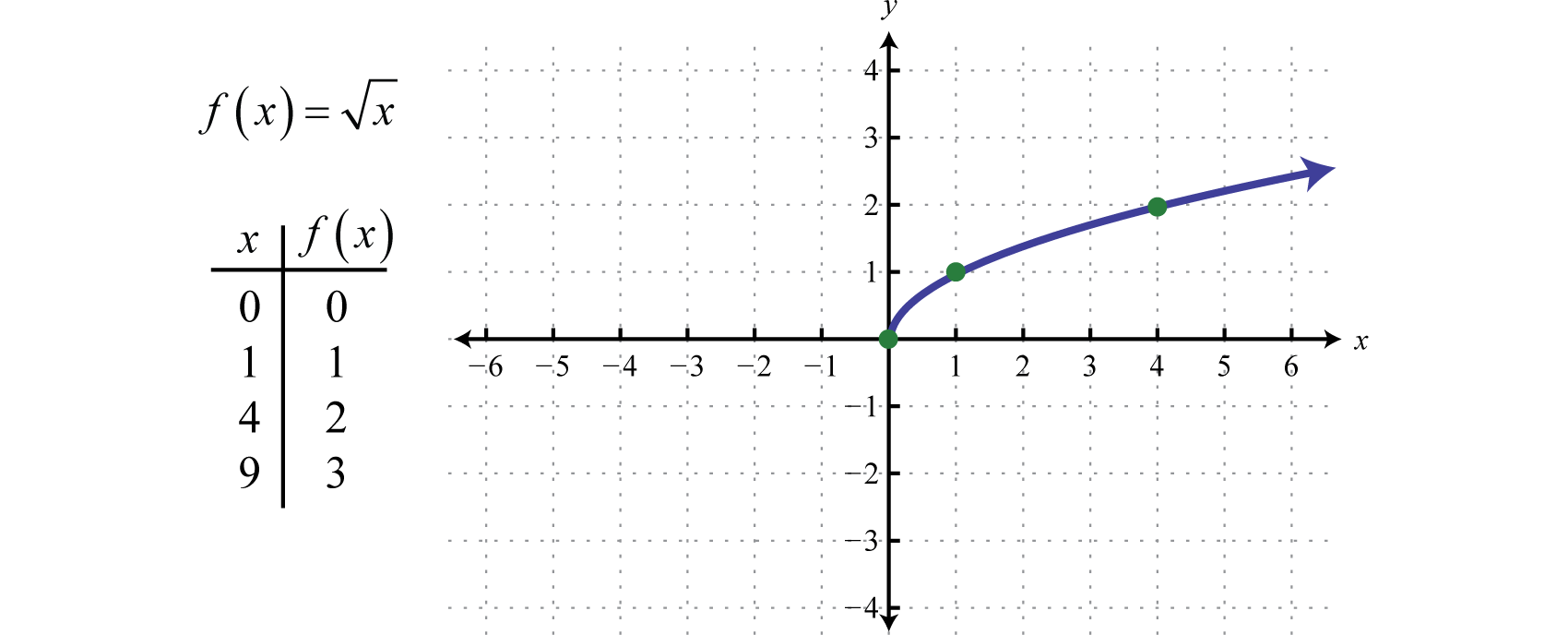The domain and range both consist of real numbers greater than or equal to zero: $[0,∞).$ To determine the domain of a function involving a square root we look at the radicand and find the values that produce nonnegative results.

### Example 3

Determine the domain of the function defined by $f(x)=2x+3.$

Solution:

Here the radicand is $2x+3.$ This expression must be zero or positive. In other words,

$2x+3≥0$

Solve for x.

$2x+3≥02x≥−3x≥−32$

Answer: Domain: $[−32,∞)$

A cube rootA number that when used as a factor with itself three times yields the original number, denoted with the symbol $3.$ of a number is a number that when multiplied by itself three times yields the original number. Furthermore, we denote a cube root using the symbol $3$, where 3 is called the indexThe positive integer n in the notation $n$ that is used to indicate an nth root.. For example,

$643=4, because 43=64$

The product of three equal factors will be positive if the factor is positive and negative if the factor is negative. For this reason, any real number will have only one real cube root. Hence the technicalities associated with the principal root do not apply. For example,

$−643=−4, because (−4)3=−64$

In general, given any real number a, we have the following property:

$a33=a if a∈ℝ$

When simplifying cube roots, look for factors that are perfect cubes.

### Example 4

Evaluate.

1. $83$
2. $03$
3. $1273$
4. $−13$
5. $−1253$

Solution:

1. $83=233=2$
2. $03=033=0$
3. $1273=(13)33=13$
4. $−13=(−1)33=−1$
5. $−1253=(−5)33=−5$

It may be the case that the radicand is not a perfect square or cube. If an integer is not a perfect power of the index, then its root will be irrational. For example, $23$ is an irrational number that can be approximated on most calculators using the root button $x.$ Depending on the calculator, we typically type in the index prior to pushing the button and then the radicand as follows:

$3 yx 2 =$

Therefore, we have

$23≈1.260, because 1.260^3≈2$

Since cube roots can be negative, zero, or positive we do not make use of any absolute values.

### Example 5

Simplify: $(y−7)33.$

Solution:

The cube root of a quantity cubed is that quantity.

$(y−7)33=y−7$

Answer: $y−7$

Try this! Evaluate: $−10003.$

Next, consider the cube root functionThe function defined by $f(x)=x3.$:

$f(x)=x3 Cube root function.$

Since the cube root could be either negative or positive, we conclude that the domain consists of all real numbers. Sketch the graph by plotting points. Choose some positive and negative values for x, as well as zero, and then calculate the corresponding y-values.

$xf(x)=x3Ordered Pairs−8−2f(−8)=−83=−2(−8,−2)−1−1f(−1)=−13=−1(−1,−1)00f(0)=03=0(0,0)11f(1)=13=1(1,1)82f(8)=83=2(8,2)$

Plot the points and sketch the graph of the cube root function.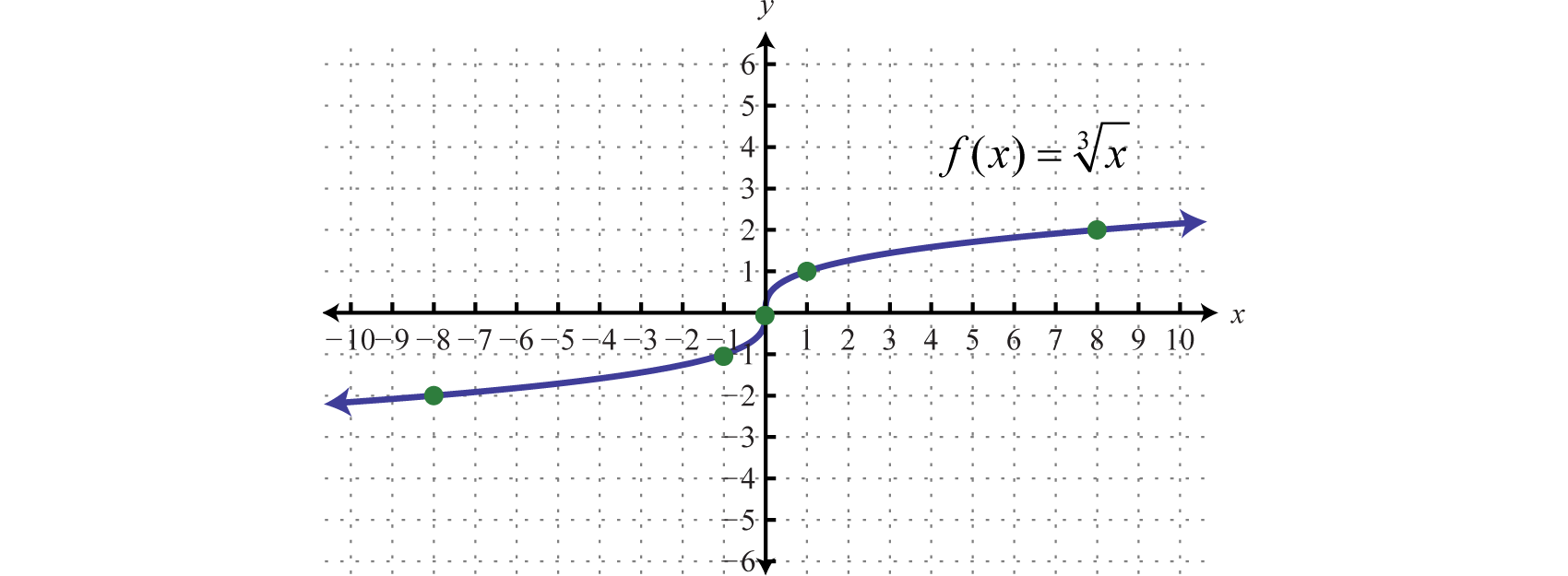The graph passes the vertical line test and is indeed a function. In addition, the range consists of all real numbers.

### Example 6

Given $g(x)=x+13+2$, find $g(−9)$, $g(−2)$, $g(−1)$, and $g(0).$ Sketch the graph of $g.$

Solution:

Replace x with the given values.

$xg(x)g(x)=x+13+2Ordered Pairs−90g(−9)=−9+13+2=−83+2=−2+2=0(−9,0)−21g(−2)=−2+13+2=−13+2=−1+2=1(−2,1)−12g(−1)=−1+13+2=03+2=0+2=2(−1,2)03g(0)=0+13+2=13+2=1+2=3(0,3)$

We can also sketch the graph using the following translations:

$y=x3Basic cube root functiony=x+13Horizontal shift left 1 unity=x+13+2Vertical shift up 2 units$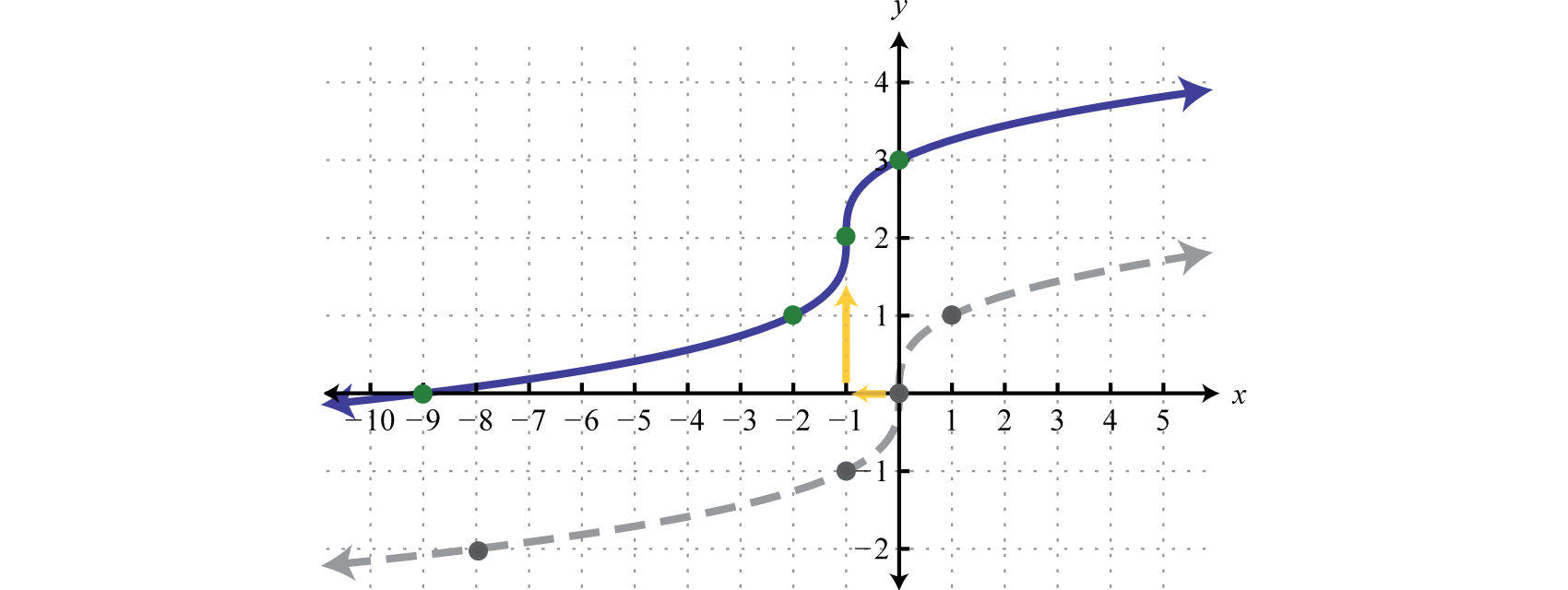## nth Roots

For any integer $n≥2$, we define an nth rootA number that when raised to the nth power $(n≥2)$ yields the original number. of a positive real number as a number that when raised to the nth power yields the original number. Given any nonnegative real number a, we have the following property:

$ann=a, if a≥0$

Here $n$ is called the index and $an$ is called the radicand. Furthermore, we can refer to the entire expression $An$ as a radicalUsed when referring to an expression of the form $An.$. When the index is an integer greater than or equal to 4, we say “fourth root,” “fifth root,” and so on. The nth root of any number is apparent if we can write the radicand with an exponent equal to the index.

### Example 7

Simplify.

1. $814$
2. $325$
3. $17$
4. $1164$

Solution:

1. $814=344=3$
2. $325=255=2$
3. $17=177=1$
4. $1164=(12)44=12$

Note: If the index is $n=2$, then the radical indicates a square root and it is customary to write the radical without the index; $a2=a.$

We have already taken care to define the principal square root of a real number. At this point, we extend this idea to nth roots when n is even. For example, 3 is a fourth root of 81, because $34=81.$ And since $(−3)4=81$, we can say that −3 is a fourth root of 81 as well. Hence we use the radical sign $n$ to denote the principal (nonnegative) nth rootThe positive nth root when n is even. when n is even. In this case, for any real number a, we use the following property:

$ann=|a| When n is even$

For example,

$814=344=|3|=3 814=(−3)44=|−3|=3$

The negative nth root, when n is even, will be denoted using a negative sign in front of the radical $− n.$

$−814=−344=−3$

We have seen that the square root of a negative number is not real because any real number that is squared will result in a positive number. In fact, a similar problem arises for any even index:

$−814=? or ( ? )4=−81$

We can see that a fourth root of −81 is not a real number because the fourth power of any real number is always positive.

$−4−814−646} These radicals are not real numbers.$

You are encouraged to try all of these on a calculator. What does it say?

### Example 8

Simplify.

1. $(−10)44$
2. $−1044$
3. $(2y+1)66$

Solution:

Since the indices are even, use absolute values to ensure nonnegative results.

1. $(−10)44=|−10|=10$
2. $−1044=−10,0004$ is not a real number.
3. $(2y+1)66=|2y+1|$

When the index n is odd, the same problems do not occur. The product of an odd number of positive factors is positive and the product of an odd number of negative factors is negative. Hence when the index n is odd, there is only one real nth root for any real number a. And we have the following property:

$ann=a When n is odd$

### Example 9

Simplify.

1. $(−10)55$
2. $−325$
3. $(2y+1)77$

Solution:

Since the indices are odd, the absolute value is not used.

1. $(−10)55=−10$
2. $−325=(−2)55=−2$
3. $(2y+1)77=2y+1$

In summary, for any real number a we have,

$ann=| a |When n is evenann=aWhen n is odd$

When n is odd, the nth root is positive or negative depending on the sign of the radicand.

$273=333 =3−273=(−3)33=−3$

When n is even, the nth root is positive or not real depending on the sign of the radicand.

$164=244=2164=(−2)44=|−2|=2−164 Not a real number$

Try this! Simplify: $−8−325.$

It will not always be the case that the radicand is a perfect power of the given index. If it is not, then we use the product rule for radicalsGiven real numbers $An$ and $Bn$, $A⋅Bn=An⋅Bn.$ and the quotient rule for radicalsGiven real numbers $An$ and $Bn$, $ABn=AnBn$ where $B≠0.$ to simplify them. Given real numbers $An$ and $Bn$,

 Product Rule for Radicals: $A⋅Bn=An⋅Bn$ Quotient Rule for Radicals: $ABn=AnBn$

A radical is simplifiedA radical where the radicand does not consist of any factors that can be written as perfect powers of the index. if it does not contain any factors that can be written as perfect powers of the index.

### Example 10

Simplify: $150.$

Solution:

Here 150 can be written as $2⋅3⋅52.$

$150=2⋅3⋅52Apply the product rule for radicals.=2⋅3⋅52Simplify.=6 ⋅ 5=56$

We can verify our answer on a calculator:

$150≈12.25 and 56≈12.25$

Also, it is worth noting that

$12.252≈150$

Answer: $56$

Note: $56$ is the exact answer and 12.25 is an approximate answer. We present exact answers unless told otherwise.

### Example 11

Simplify: $1603.$

Solution:

Use the prime factorization of 160 to find the largest perfect cube factor:

$160=25⋅5=23⋅22⋅5$

Replace the radicand with this factorization and then apply the product rule for radicals.

$1603=23⋅22⋅53Apply the product rule for radicals.=233⋅22⋅53Simplify.=2⋅203$

We can verify our answer on a calculator.

$1603≈5.43 and 2203≈5.43$

Answer: $2203$

### Example 12

Simplify: $−3205.$

Solution:

Here we note that the index is odd and the radicand is negative; hence the result will be negative. We can factor the radicand as follows:

$−320=−1⋅32⋅10=(−1)5⋅(2)5⋅10$

Then simplify:

$−3205=(−1)5⋅(2)5⋅105Apply the product rule for radicals.=(−1)55⋅(2)55⋅105Simplify.=−1⋅2⋅105=−2⋅105$

Answer: $−2105$

### Example 13

Simplify: $−8643.$

Solution:

In this case, consider the equivalent fraction with $−8=(−2)3$ in the numerator and $64=43$ in the denominator and then simplify.

$−8643= −864 3Apply the quotient rule for radicals.=(−2)33433Simplify.=−24=−12$

Answer: $−12$

Try this! Simplify: $80814$

Answer: $2543$

### Key Takeaways

• To simplify a square root, look for the largest perfect square factor of the radicand and then apply the product or quotient rule for radicals.
• To simplify a cube root, look for the largest perfect cube factor of the radicand and then apply the product or quotient rule for radicals.
• When working with nth roots, n determines the definition that applies. We use $ann=a$ when n is odd and $ann=|a|$ when n is even.
• To simplify nth roots, look for the factors that have a power that is equal to the index n and then apply the product or quotient rule for radicals. Typically, the process is streamlined if you work with the prime factorization of the radicand.

### Part A: Square and Cube Roots

Simplify.

1. $36$

2. $100$

3. $49$
4. $164$
5. $−16$

6. $−1$

7. $(−5)2$

8. $(−1)2$

9. $−4$

10. $−52$

11. $−(−3)2$

12. $−(−4)2$

13. $x2$

14. $(−x)2$

15. $(x−5)2$

16. $(2x−1)2$

17. $643$

18. $2163$

19. $−2163$

20. $−643$

21. $−83$

22. $13$

23. $−(−2)33$

24. $−(−7)33$

25. $183$
26. $8273$
27. $(−y)33$

28. $−y33$

29. $(y−8)33$

30. $(2x−3)33$

Determine the domain of the given function.

1. $g(x)=x+5$

2. $g(x)=x−2$

3. $f(x)=5x+1$

4. $f(x)=3x+4$

5. $g(x)=−x+1$

6. $g(x)=−x−3$

7. $h(x)=5−x$

8. $h(x)=2−3x$

9. $g(x)=x+43$

10. $g(x)=x−33$

Evaluate given the function definition.

1. Given $f(x)=x−1$, find $f(1)$, $f(2)$, and $f(5)$

2. Given $f(x)=x+5$, find $f(−5)$, $f(−1)$, and $f(20)$

3. Given $f(x)=x+3$, find $f(0)$, $f(1)$, and $f(16)$

4. Given $f(x)=x−5$, find $f(0)$, $f(1)$, and $f(25)$

5. Given $g(x)=x3$, find $g(−1)$, $g(0)$, and $g(1)$

6. Given $g(x)=x3−2$, find $g(−1)$, $g(0)$, and $g(8)$

7. Given $g(x)=x+73$, find $g(−15)$, $g(−7)$, and $g(20)$

8. Given $g(x)=x−13+2$, find $g(0)$, $g(2)$, and $g(9)$

Sketch the graph of the given function and give its domain and range.

1. $f(x)=x+9$

2. $f(x)=x−3$

3. $f(x)=x−1+2$

4. $f(x)=x+1+3$

5. $g(x)=x−13$

6. $g(x)=x+13$

7. $g(x)=x3−4$

8. $g(x)=x3+5$

9. $g(x)=x+23−1$

10. $g(x)=x−23+3$

11. $f(x)=−x3$

12. $f(x)=−x−13$

### Part B: nth Roots

Simplify.

1. $644$

2. $164$

3. $6254$

4. $14$

5. $2564$

6. $10,0004$

7. $2435$

8. $100,0005$

9. $1325$
10. $12435$
11. $−164$

12. $−16$

13. $−325$

14. $−15$

15. $−1$

16. $−164$

17. $−6−273$

18. $−5−83$

19. $2−1,0003$

20. $7−2435$

21. $6−164$

22. $12−646$

23. $32516$
24. $6169$
25. $5271253$
26. $732755$
27. $−58273$
28. $−8625164$
29. $2100,0005$

30. $21287$

Simplify.

1. $96$

2. $500$

3. $480$

4. $450$

5. $320$

6. $216$

7. $5112$

8. $10135$

9. $−2240$

10. $−3162$

11. $15049$
12. $2009$
13. $675121$
14. $19281$
15. $543$

16. $243$

17. $483$

18. $813$

19. $403$

20. $1203$

21. $1623$

22. $5003$

23. $541253$
24. $403433$
25. $5−483$

26. $2−1083$

27. $8964$

28. $71624$

29. $1605$

30. $4865$

31. $2242435$
32. $5325$
33. $−1325$
34. $−1646$

Simplify. Give the exact answer and the approximate answer rounded to the nearest hundredth.

1. $60$

2. $600$

3. $9649$
4. $19225$
5. $2403$

6. $3203$

7. $2881253$
8. $62583$
9. $4864$

10. $2885$

Rewrite the following as a radical expression with coefficient 1.

1. $215$

2. $37$

3. $510$

4. $103$

5. $273$

6. $363$

7. $254$

8. $324$

9. Each side of a square has a length that is equal to the square root of the square’s area. If the area of a square is 72 square units, find the length of each of its sides.

10. Each edge of a cube has a length that is equal to the cube root of the cube’s volume. If the volume of a cube is 375 cubic units, find the length of each of its edges.

11. The current I measured in amperes is given by the formula $I=PR$ where P is the power usage measured in watts and R is the resistance measured in ohms. If a 100 watt light bulb has 160 ohms of resistance, find the current needed. (Round to the nearest hundredth of an ampere.)

12. The time in seconds an object is in free fall is given by the formula $t=s4$ where s represents the distance in feet the object has fallen. How long will it take an object to fall to the ground from the top of an 8-foot stepladder? (Round to the nearest tenth of a second.)

### Part D: Discussion Board

1. Explain why there are two real square roots for any positive real number and one real cube root for any real number.

2. What is the square root of 1 and what is the cube root of 1? Explain why.

3. Explain why $−1$ is not a real number and why $−13$ is a real number.

4. Research and discuss the methods used for calculating square roots before the common use of electronic calculators.

1. 6

2. $23$

3. −4

4. 5

5. Not a real number

6. −3

7. $|x|$

8. $|x−5|$

9. 4

10. −6

11. −2

12. 2

13. $12$

14. $−y$

15. $y−8$

16. $[−5,∞)$

17. $[−15,∞)$
18. $(−∞,1]$

19. $(−∞,5]$

20. $(−∞,∞)$

21. $f(1)=0$; $f(2)=1$; $f(5)=2$

22. $f(0)=3$; $f(1)=4$; $f(16)=7$

23. $g(−1)=−1$; $g(0)=0$; $g(1)=1$

24. $g(−15)=−2$; $g(−7)=0$; $g(20)=3$

25. Domain: $[−9,∞)$; range: $[0,∞)$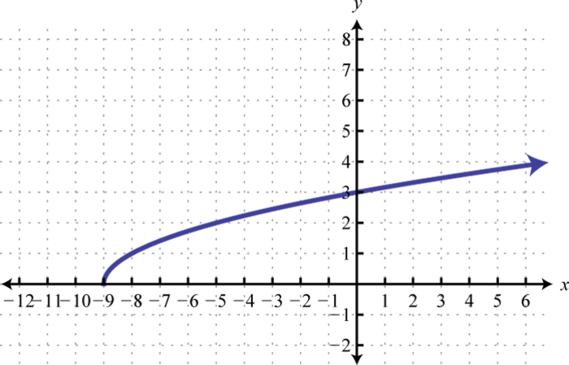26. Domain: $[1,∞)$; range: $[2,∞)$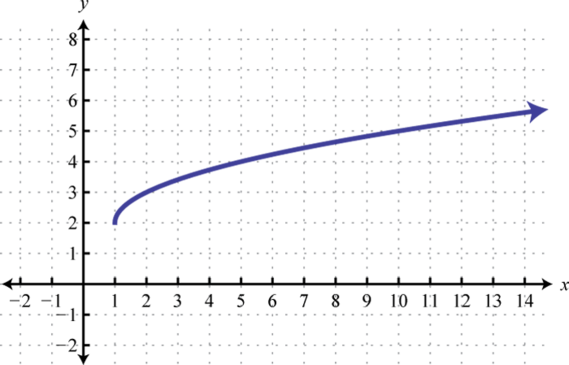27. Domain: $ℝ$; range: $ℝ$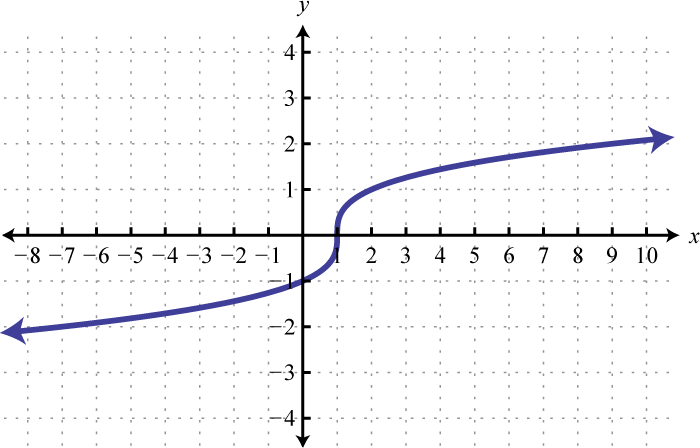28. Domain: $ℝ$; range: $ℝ$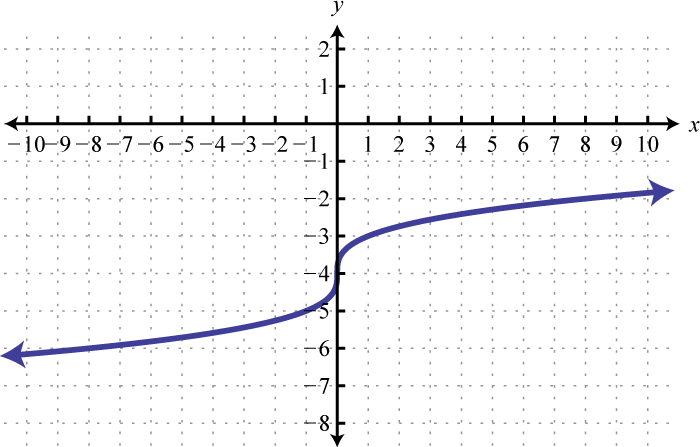29. Domain: $ℝ$; range: $ℝ$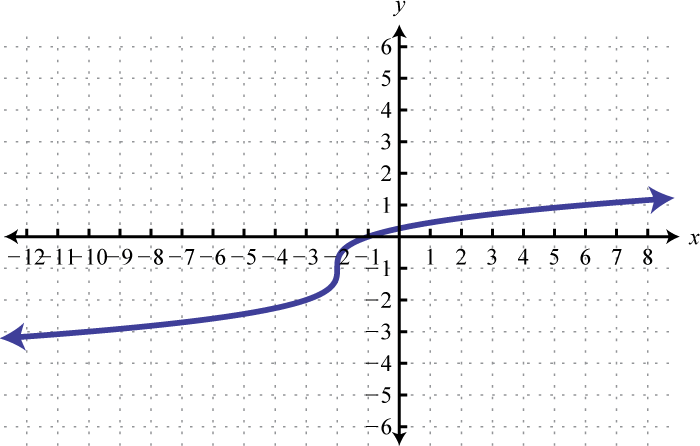30. Domain: $ℝ$; range: $ℝ$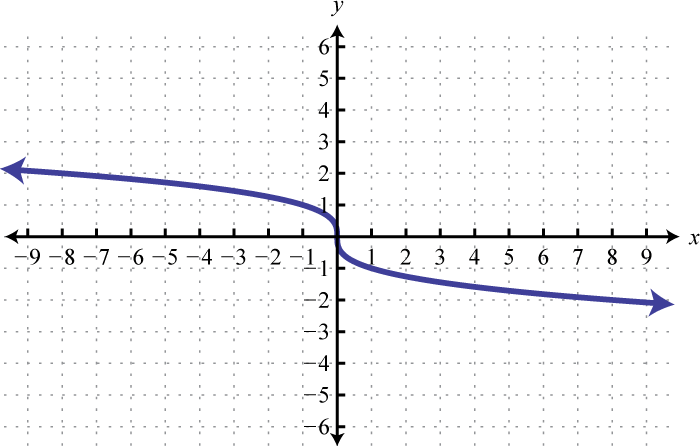1. 4

2. 5

3. 4

4. 3

5. $12$

6. −2

7. −2

8. Not a real number

9. 18

10. −20

11. Not a real number

12. $154$

13. 3

14. $−103$

15. 20

1. $46$

2. $430$

3. $85$

4. $207$

5. $−815$

6. $567$
7. $15311$
8. $323$

9. $263$

10. $253$

11. $3 63$

12. $3235$
13. $−1063$

14. $1664$

15. $255$

16. $2753$
17. $−12$

18. $215$; 7.75

19. $467$; 1.40

20. $2303$; 6.21

21. $23635$; 1.32

22. $364$; 4.70

23. $60$

24. $250$

25. $563$

26. $804$

27. $62$ units

### Learning Objectives

1. Simplify radical expressions using the product and quotient rule for radicals.

An algebraic expression that contains radicals is called a radical expressionAn algebraic expression that contains radicals.. We use the product and quotient rules to simplify them.

### Example 1

Simplify: $27x33.$

Solution:

Use the fact that $ann=a$ when n is odd.

$27x33=33⋅x33Apply the product rule for radicals.=333⋅x33Simplify.=3⋅x=3x$

Answer: $3x$

### Example 2

Simplify: $16y44.$

Solution:

Use the fact that $ann=|a|$ when n is even.

$16y44=24y44Apply the product rule for radicals.=244⋅y44Simplify.=2⋅|y|=2|y|$

Since y is a variable, it may represent a negative number. Thus we need to ensure that the result is positive by including the absolute value.

Answer: $2|y|$

### Important Note

Typically, at this point in algebra we note that all variables are assumed to be positive. If this is the case, then y in the previous example is positive and the absolute value operator is not needed. The example can be simplified as follows.

$16y44=24y44 =244⋅y44=2y$

In this section, we will assume that all variables are positive. This allows us to focus on calculating nth roots without the technicalities associated with the principal nth root problem. For this reason, we will use the following property for the rest of the section,

$ann=a, if a≥0 nth root$

When simplifying radical expressions, look for factors with powers that match the index.

### Example 3

Simplify: $12x6y3.$

Solution:

Begin by determining the square factors of 12, $x6$, and $y3.$

$12=22⋅3x6=(x3)2 y3=y2⋅y } Square factors$

Make these substitutions, and then apply the product rule for radicals and simplify.

$12x6y3=22⋅3⋅(x3)2⋅y2⋅yApply the product rule for radicals.=22⋅(x3)2⋅y2⋅3ySimplify.=2 ⋅ x3 ⋅ y ⋅3y=2x3y3y$

Answer: $2x3y3y$

### Example 4

Simplify: $18a5b8$.

Solution:

Begin by determining the square factors of 18, $a5$, and $b8.$

$18=2⋅32a5=a2⋅a2⋅a=(a2)2⋅a b8=b4⋅b4=(b4)2 } Square factors$

Make these substitutions, apply the product and quotient rules for radicals, and then simplify.

$18a5b8=2⋅32⋅(a2)2⋅a(b4)2Apply the product and quotient rule for radicals.=32⋅(a2)2⋅2a(b4)2Simplify.= 3a22ab4$

Answer: $3a22ab4$

### Example 5

Simplify: $80x5y73.$

Solution:

Begin by determining the cubic factors of 80, $x5$, and $y7.$

$80=24⋅5=23⋅2⋅5x5=x3⋅x2y7=y6⋅y=(y2)3⋅y } Cubic factors$

Make these substitutions, and then apply the product rule for radicals and simplify.

$80x5y73=23⋅2⋅5⋅x3⋅x2⋅(y2)3⋅y3 =233⋅x33⋅(y2)33⋅2⋅5⋅x2⋅y3=2⋅xy2⋅10x2y3=2xy2 10x2y3$

Answer: $2xy210x2y3$

### Example 6

Simplify $9x6y3z93$.

Solution:

The coefficient $9=32$, and thus does not have any perfect cube factors. It will be left as the only remaining radicand because all of the other factors are cubes, as illustrated below:

$x6=(x2)3y3=(y)3z9=(z3)3 } Cubic factors$

Replace the variables with these equivalents, apply the product and quotient rules for radicals, and then simplify.

$9x6y3z93=9⋅(x2)3y3⋅(z3)33=93⋅(x2)33y33⋅(z3)33=93⋅x2y⋅z3=x2 93yz3$

Answer: $x293yz3$

### Example 7

Simplify: $81a4b54.$

Solution:

Determine all factors that can be written as perfect powers of 4. Here, it is important to see that $b5=b4⋅b.$ Hence the factor $b$ will be left inside the radical.

$81a4b54=34⋅a4⋅b4⋅b4=344⋅a44⋅b44⋅b4=3⋅a⋅b⋅b4=3abb4$

Answer: $3abb4$

### Example 8

Simplify: $−32x3y6z55.$

Solution:

Notice that the variable factor x cannot be written as a power of 5 and thus will be left inside the radical. In addition, $y6=y5⋅y$; the factor y will be left inside the radical as well.

$−32x3y6z55=(−2)5⋅x3⋅y5⋅y⋅z55=(−2)55⋅y55⋅z55⋅x3⋅y5=−2⋅y⋅z⋅x3⋅y5=−2yzx3y5$

Answer: $−2yzx3y5$

Tip: To simplify finding an nth root, divide the powers by the index.

$a6=a3, which is a6÷2=a3b63=b2, which is b6÷3=b2c66=c , which is c6÷6=c1$

If the index does not divide into the power evenly, then we can use the quotient and remainder to simplify. For example,

$a5=a2⋅a, which is a5÷2=a2 r 1b53=b⋅b23, which is b5÷3=b1 r 2c145=c2⋅c45, which is c14÷5=c2 r 4$

The quotient is the exponent of the factor outside of the radical, and the remainder is the exponent of the factor left inside the radical.

Try this! Simplify: $162a7b5c43.$

Answer: $3a2bc6ab2c3$

Formulas often consist of radical expressions. For example, the period of a pendulum, or the time it takes a pendulum to swing from one side to the other and back, depends on its length according to the following formula.

$T=2πL32$

Here T represents the period in seconds and L represents the length in feet of the pendulum.

### Example 9

If the length of a pendulum measures $112$ feet, then calculate the period rounded to the nearest tenth of a second.

Solution:

Substitute $112=32$ for L and then simplify.

$T=2πL32=2π3232=2π32⋅132Apply the quotient rule for radicals.=2π364Simplify.=2π38 =π34≈1.36$

Answer: The period is approximately 1.36 seconds.

Frequently you need to calculate the distance between two points in a plane. To do this, form a right triangle using the two points as vertices of the triangle and then apply the Pythagorean theorem. Recall that the Pythagorean theorem states that if given any right triangle with legs measuring a and b units, then the square of the measure of the hypotenuse c is equal to the sum of the squares of the legs: $a2+b2=c2.$ In other words, the hypotenuse of any right triangle is equal to the square root of the sum of the squares of its legs.### Example 10

Find the distance between (−5, 3) and (1, 1).

Solution:

Form a right triangle by drawing horizontal and vertical lines though the two points. This creates a right triangle as shown below: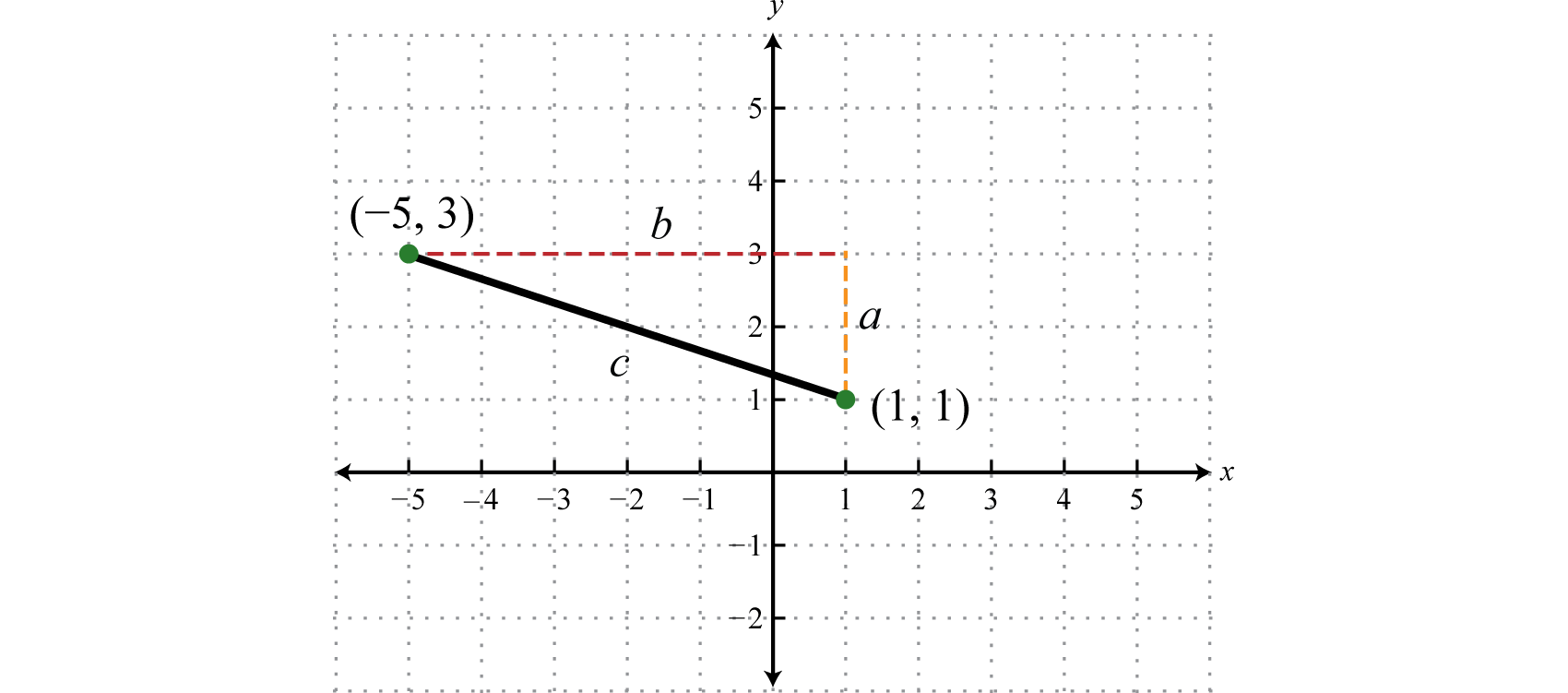The length of leg b is calculated by finding the distance between the x-values of the given points, and the length of leg a is calculated by finding the distance between the given y-values.

$a=3−1=2 unitsb=1−(−5)=1+5=6 units$

Next, use the Pythagorean theorem to find the length of the hypotenuse.

$c=22+62=4+36=40=4⋅10=210 units$

Answer: The distance between the two points is $210$ units.

Generalize this process to produce a formula that can be used to algebraically calculate the distance between any two given points.Given two points, $( x1, y1)$ and $( x2, y2)$, the distance, d, between them is given by the distance formulaGiven two points $(x1, y1)$ and $(x2, y2)$, calculate the distance d between them using the formula $d=(x2−x1)2+(y2−y1)2.$, $d=(x2−x1)2+(y2−y1)2.$

### Example 11

Calculate the distance between (−4, 7) and (2, 1).

Solution:

Use the distance formula with the following points.

$( x1, y1) ( x2, y2)(−4,7)(2, 1)$

It is a good practice to include the formula in its general form before substituting values for the variables; this improves readability and reduces the probability of making errors.

$d=(x2−x1)2+(y2−y1)2=(2−(−4))2+(1−7)2=(2+4)2+(1−7)2=(6)2+(−6)2=36+36=72=36⋅2=62$

Answer: The distance between the two points is $62$ units.

### Example 12

Do the three points (2, −1), (3, 2), and (8, −3) form a right triangle?

Solution:

The Pythagorean theorem states that having side lengths that satisfy the property $a2+b2=c2$ is a necessary and sufficient condition of right triangles. In other words, if you can show that the sum of the squares of the leg lengths of the triangle is equal to the square of the length of the hypotenuse, then the triangle must be a right triangle. First, calculate the length of each side using the distance formula.

Geometry

Calculation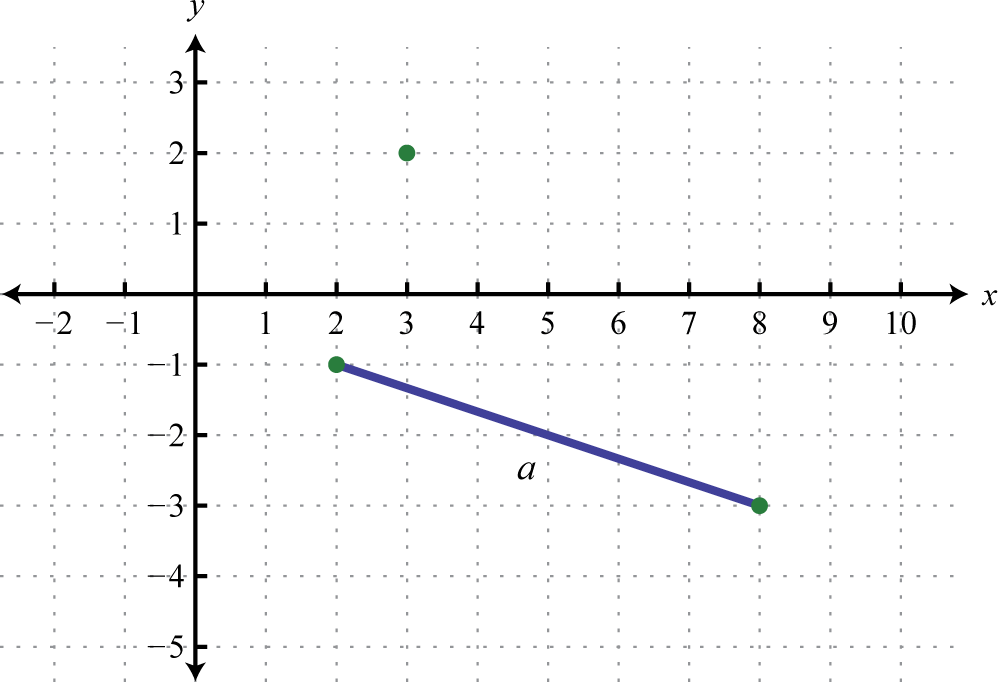Points: (2, −1) and (8, −3)

$a=(8−2)2+[−3−(−1)]2=(6)2+(−3+1)2=36+(−2)2=36+4=40=210$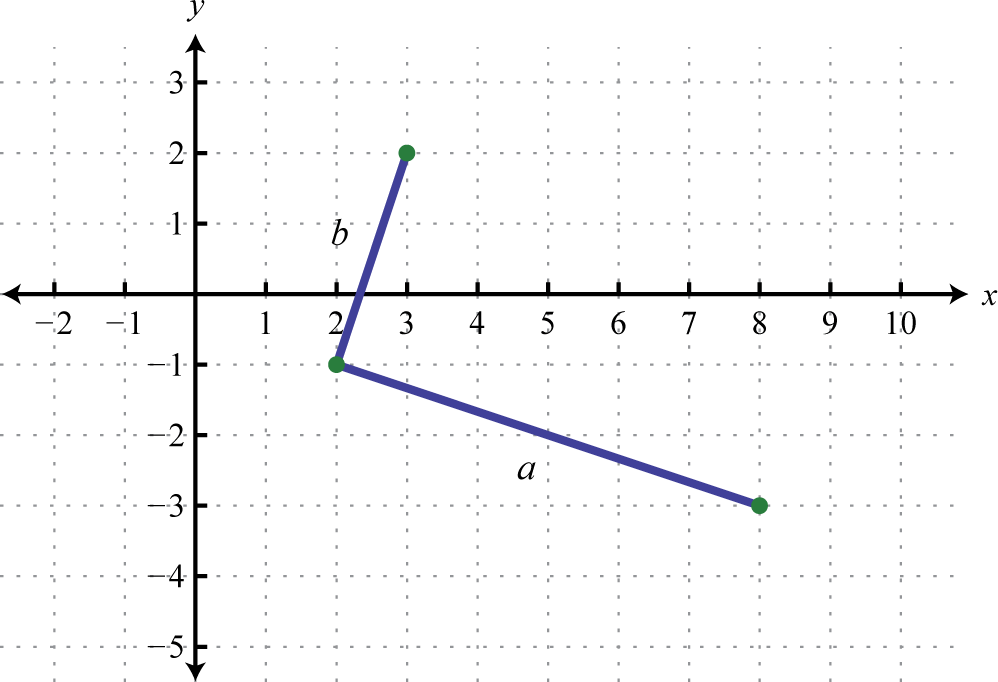Points: (2, −1) and (3, 2)

$b=(3−2)2+[2−(−1)]2=(1)2+(2+1)2=1+(3)2=1+9=10$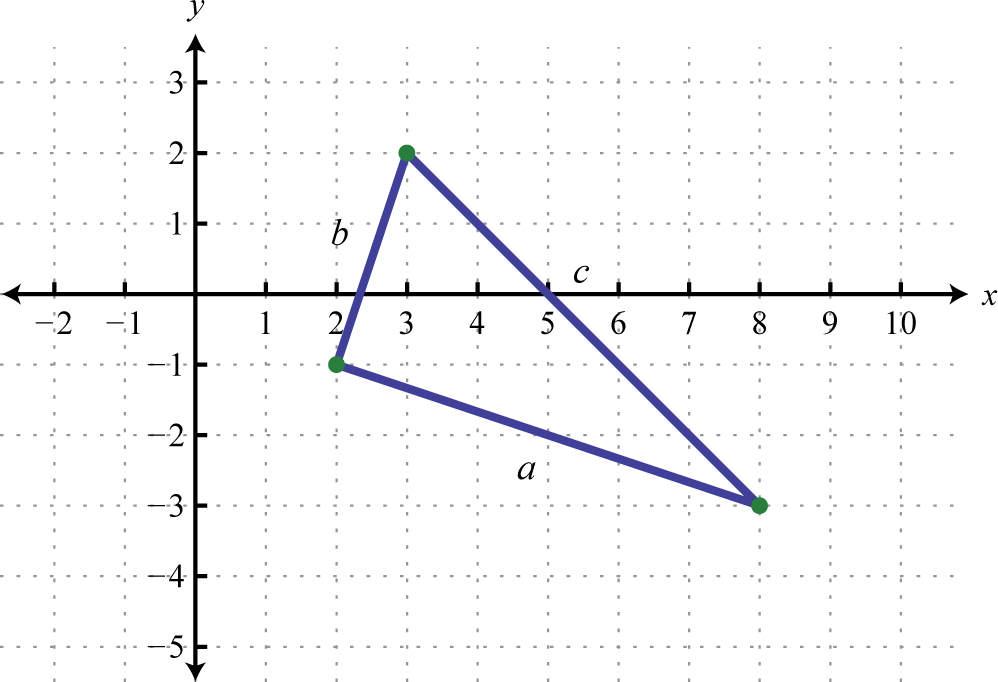Points: (3, 2) and (8, −3)

$c=(8−3)2+(−3−2)2=(5)2+(−5)2=25+25=50=52$

Now we check to see if $a2+b2=c2.$

$a2+b2=c2(210)2+(10)2=(52)24(10)2+(10)2=25(2)24⋅10+10=25⋅250=50 ✓$

Answer: Yes, the three points form a right triangle.

Try this! The speed of a vehicle before the brakes were applied can be estimated by the length of the skid marks left on the road. On wet concrete, the speed v in miles per hour can be estimated by the formula $v=23d$, where d represents the length of the skid marks in feet. Estimate the speed of a vehicle before applying the brakes if the skid marks left behind measure 27 feet. Round to the nearest mile per hour.

### Key Takeaways

• To simplify a radical expression, look for factors of the radicand with powers that match the index. If found, they can be simplified by applying the product and quotient rules for radicals, as well as the property $ann=a$, where a is nonnegative.
• A radical expression is simplified if its radicand does not contain any factors that can be written as perfect powers of the index.
• We typically assume that all variable expressions within the radical are nonnegative. This allows us to focus on simplifying radicals without the technical issues associated with the principal nth root. If this assumption is not made, we will ensure a positive result by using absolute values when simplifying radicals with even indices.

### Part A: Simplifying Radical Expressions

Assume that the variable could represent any real number and then simplify.

1. $9x2$

2. $16y2$

3. $8y33$

4. $125a33$

5. $64x44$

6. $81y44$

7. $36a4$

8. $100a8$

9. $4a6$

10. $a10$

11. $18a4b5$

12. $48a5b3$

13. $128x6y86$

14. $a6b7c86$

15. $(5x−4)2$

16. $(3x−5)4$

17. $x2−6x+9$

18. $x2−10x+25$

19. $4x2+12x+9$

20. $9x2+6x+1$

Simplify. (Assume all variable expressions represent positive numbers.)

1. $49a2$

2. $64b2$

3. $x2y2$

4. $25x2y2z2$

5. $180x3$

6. $150y3$

7. $49a3b2$

8. $4a4b3c$

9. $45x5y3$

10. $50x6y4$

11. $64r2s6t5$

12. $144r8s6t2$

13. $(x+1)2$

14. $(2x+3)2$

15. $4(3x−1)2$

16. $9(2x+3)2$

17. $9x325y2$
18. $4x59y4$
19. $m736n4$
20. $147m9n6$
21. $2r2s525t4$
22. $36r5s2t6$
23. $27a33$

24. $125b33$

25. $250x4y33$

26. $162a3b53$

27. $64x3y6z93$

28. $216x12y33$

29. $8x3y43$

30. $27x5y33$

31. $a4b5c63$

32. $a7b5c33$

33. $8x427y33$
34. $x5125y63$
35. $360r5s12t133$

36. $540r3s2t93$

37. $81x44$

38. $x4y44$

39. $16x4y84$

40. $81x12y44$

41. $a4b5c64$

42. $54a6c84$

43. $128x64$

44. $243y74$

45. $32m10n55$
46. $37m9n105$
47. $−34x2$

48. $79y2$

49. $−5x4x2y$

50. $−3y16x3y2$

51. $12aba5b3$

52. $6a2b9a7b2$

53. $2x8x63$

54. $−5x227x33$

55. $2ab−8a4b53$

56. $5a2b−27a3b33$

Rewrite the following as a radical expression with coefficient 1.

1. $3x6x$

2. $5y5y$

3. $ab10a$

4. $2ab2a$

5. $m2nmn$

6. $2m2n33n$

7. $2x3x3$

8. $3yy23$

9. $2y24y4$

10. $x2y9xy25$

### Part B: Formulas Involving Radicals

The period T in seconds of a pendulum is given by the formula $T=2πL32$ where L represents the length in feet of the pendulum. Calculate the period, given each of the following lengths. Give the exact value and the approximate value rounded to the nearest tenth of a second.

1. 8 feet

2. 32 feet

3. $12$ foot

4. $18$ foot

The time t in seconds an object is in free fall is given by the formula $t=s4$ where s represents the distance in feet the object has fallen. Calculate the time it takes an object to fall, given each of the following distances. Give the exact value and the approximate value rounded to the nearest tenth of a second.

1. 48 feet

2. 80 feet

3. 192 feet

4. 288 feet

5. The speed of a vehicle before the brakes were applied can be estimated by the length of the skid marks left on the road. On dry pavement, the speed v in miles per hour can be estimated by the formula $v=26d$, where d represents the length of the skid marks in feet. Estimate the speed of a vehicle before applying the brakes on dry pavement if the skid marks left behind measure 27 feet. Round to the nearest mile per hour.

6. The radius r of a sphere can be calculated using the formula $r=6π2V32π$, where V represents the sphere’s volume. What is the radius of a sphere if the volume is $36π$ cubic centimeters?

Given the function find the y-intercept

1. $f(x)=x+12$

2. $f(x)=x+8−3$

3. $f(x)=x−83$

4. $f(x)=x+273$

5. $f(x)=x+163$

6. $f(x)=x+33−1$

Use the distance formula to calculate the distance between the given two points.

1. (5, −7) and (3, −8)

2. (−9, 7) and (−8, 4)

3. (−3, −4) and (3, −6)

4. (−5, −2) and (1, −6)

5. (−1, 1) and (−4, 10)

6. (8, −3) and (2, −12)

7. (0, −6) and (−3, 0)

8. (0, 0) and (8, −4)

9. $(12,−12)$ and $(−1,32)$

10. $(−13,2)$ and $(53,−23)$

Determine whether or not the three points form a right triangle. Use the Pythagorean theorem to justify your answer.

1. (2,−1), (−1,2), and (6,3)

2. (−5,2), (−1, −2), and (−2,5)

3. (−5,0), (0,3), and (6,−1)

4. (−4,−1), (−2,5), and (7,2)

5. (1,−2), (2,3), and (−3,4)

6. (−2,1), (−1,−1), and (1,3)

7. (−4,0), (−2,−10), and (3,−9)

8. (0,0), (2,4), and (−2,6)

### Part D: Discussion Board

1. Give a value for x such that $x2≠x.$ Explain why it is important to assume that the variables represent nonnegative numbers.

2. Research and discuss the accomplishments of Christoph Rudolff. What is he credited for?

3. What is a surd, and where does the word come from?

4. Research ways in which police investigators can determine the speed of a vehicle after an accident has occurred. Share your findings on the discussion board.

1. $3|x|$

2. $2y$

3. $2|x|$

4. $6a2$

5. $2|a3|$
6. $3a2b22b$

7. $2|xy|2y26$

8. $|5x−4|$

9. $|x−3|$

10. $|2x+3|$

11. $7a$

12. $xy$

13. $6x5x$

14. $7aba$

15. $3x2y5xy$

16. $8rs3t2t$

17. $x+1$

18. $2(3x−1)$

19. $3xx5y$
20. $m3m6n2$
21. $rs22s5t2$
22. $3a$

23. $5xy2x3$

24. $4xy2z3$

25. $2xyy3$

26. $abc2ab23$

27. $2xx33y$
28. $2rs4t445r2t3$

29. $3x$

30. $2xy2$

31. $abcbc24$

32. $2x8x24$

33. $2m2n$
34. $−6x$

35. $−10x2y$

36. $12a3b2ab$

37. $4x3$

38. $−4a2b2ab23$

39. $54x3$

40. $10a3b2$

41. $m5n3$

42. $24x43$

43. $64y94$

1. $π$ seconds; 3.1 seconds

2. $π4$ seconds; 0.8 seconds

3. $3$ seconds; 1.7 seconds

4. $23$ seconds; 3.5 seconds

5. 25 miles per hour

6. $(0,23)$

7. $(0,−2)$

8. $(0,223)$
9. $5$ units

10. $210$ units

11. $310$ units

12. $35$ units

13. $52$ units

14. Right triangle

15. Not a right triangle

16. Right triangle

17. Right triangle

### Learning Objectives

Adding and subtracting radical expressions is similar to adding and subtracting like terms. Radicals are considered to be like radicalsRadicals that share the same index and radicand., or similar radicalsTerm used when referring to like radicals., when they share the same index and radicand. For example, the terms $26$ and $56$ contain like radicals and can be added using the distributive property as follows:

$26+56=(2+5)6=76$

Typically, we do not show the step involving the distributive property and simply write,

$26+56=76$

### Example 1

Add: $753+353.$

Solution:

$753+353=1053$

Answer: $1053$

Subtraction is performed in a similar manner.

### Example 2

Subtract: $410−510.$

Solution:

$410−510=(4−5)10=−110=−10$

Answer: $−10$

If the radicand and the index are not exactly the same, then the radicals are not similar and we cannot combine them.

### Example 3

Simplify: $105+62−95−72.$

Solution:

$105+62−95−72=105−95+62−72= 5 − 2$

We cannot simplify any further because $5$ and $2$ are not like radicals; the radicands are not the same.

Answer: $5−2$

$Caution:$ It is important to point out that $5−2≠5−2.$ We can verify this by calculating the value of each side with a calculator.

$5−2≈0.825−2=3≈1.73$

In general, note that $an±bn≠a±bn.$

### Example 4

Simplify: $5103+310−103−210.$

Solution:

$5 103+310−103−210=5 103−103+310−210= 4 103 + 10$

We cannot simplify any further, because $103$ and $10$ are not like radicals; the indices are not the same.

Answer: $4103+10$

Often, we will have to simplify before we can identify the like radicals within the terms.

### Example 5

Subtract: $32−18+50.$

Solution:

At first glance, the radicals do not appear to be similar. However, after simplifying completely, we will see that we can combine them.

$32−18+50=16⋅2−9⋅2+25⋅2=42−32+52=62$

Answer: $62$

### Example 6

Simplify: $1083+243−323−813.$

Solution:

Begin by looking for perfect cube factors of each radicand.

$1083+243−323−813=27⋅43+8⋅33−8⋅43−27⋅33Simplify.=343+233−243−333Combine like radicals.=43−33$

Answer: $43−33$

Try this! Simplify: $20+27−35−212.$

Answer: $−5−3$

Next, we work with radical expressions involving variables. In this section, assume all radicands containing variable expressions are nonnegative.

### Example 7

Simplify: $−95x3−2x3+105x3.$

Solution:

$−95x3−2x3+105x3=−9 5x3+10 5x3−2x3=5x3−2x3$

We cannot combine any further because the remaining radical expressions do not share the same radicand; they are not like radicals. Note: $5x3−2x3≠5x−2x3.$

Answer: $5x3−2x3$

We will often find the need to subtract a radical expression with multiple terms. If this is the case, remember to apply the distributive property before combining like terms.

### Example 8

Simplify: $(5x−4y)−(4x−7y).$

Solution:

$(5x−4y)−(4x−7y)=5x−4y−4x+7yDistribute.=5x−4x−4y+7y=x+3y$

Answer: $x+3y$

Until we simplify, it is often unclear which terms involving radicals are similar. The general steps for simplifying radical expressions are outlined in the following example.

### Example 9

Simplify: $53x43+24x33−(x 24x3+4​ ​​3x33).$

Solution:

Step 1: Simplify the radical expression. In this case, distribute and then simplify each term that involves a radical.

$53x43+24x33−(x 24x3+43x33)=53x43+24x33−x 24x3−43x33=53⋅x⋅x33+8⋅3⋅x33−x 8⋅3x3−43x33=5x 3x3+2x 33−2x 3x3−4x 33$

Step2: Combine all like radicals. Remember to add only the coefficients; the variable parts remain the same.

$=5x 3x3+2x33−2x 3x3−4​x ​​33=3x 3x3−2x33$

Answer: $3x3x3−2x33$

### Example 10

Simplify: $2a125a2b−a280b+420a4b.$

Solution:

$2a125a2b−a280b+420a4b=2a25⋅5⋅a2⋅b−a216⋅5⋅b+44⋅5⋅(a2)2bFactor.=2a⋅5⋅a5b−a2⋅45b+4⋅2⋅a25bSimplify.=10a25b−4a25b+8a25bCombine like terms.=14a25b$

Answer: $14a25b$

Try this! $2x6y3+xy33−(y 27x3−2x2x3y3)$

Answer: $3x22y3−2yx3$

### Tip

Take careful note of the differences between products and sums within a radical. Assume both x and y are nonnegative.

$Products Sums x2y2=xyx3y33=xyx2+y2≠x+yx3+y33≠x+y$

The property $a⋅bn=an⋅bn$ says that we can simplify radicals when the operation in the radicand is multiplication. There is no corresponding property for addition.

### Example 11

Calculate the perimeter of the triangle formed by the points $(−2,−1)$, $(−3,6)$, and $(2,1).$

Solution:

The formula for the perimeter of a triangle is $P=a+b+c$ where a, b, and c represent the lengths of each side. Plotting the points we have,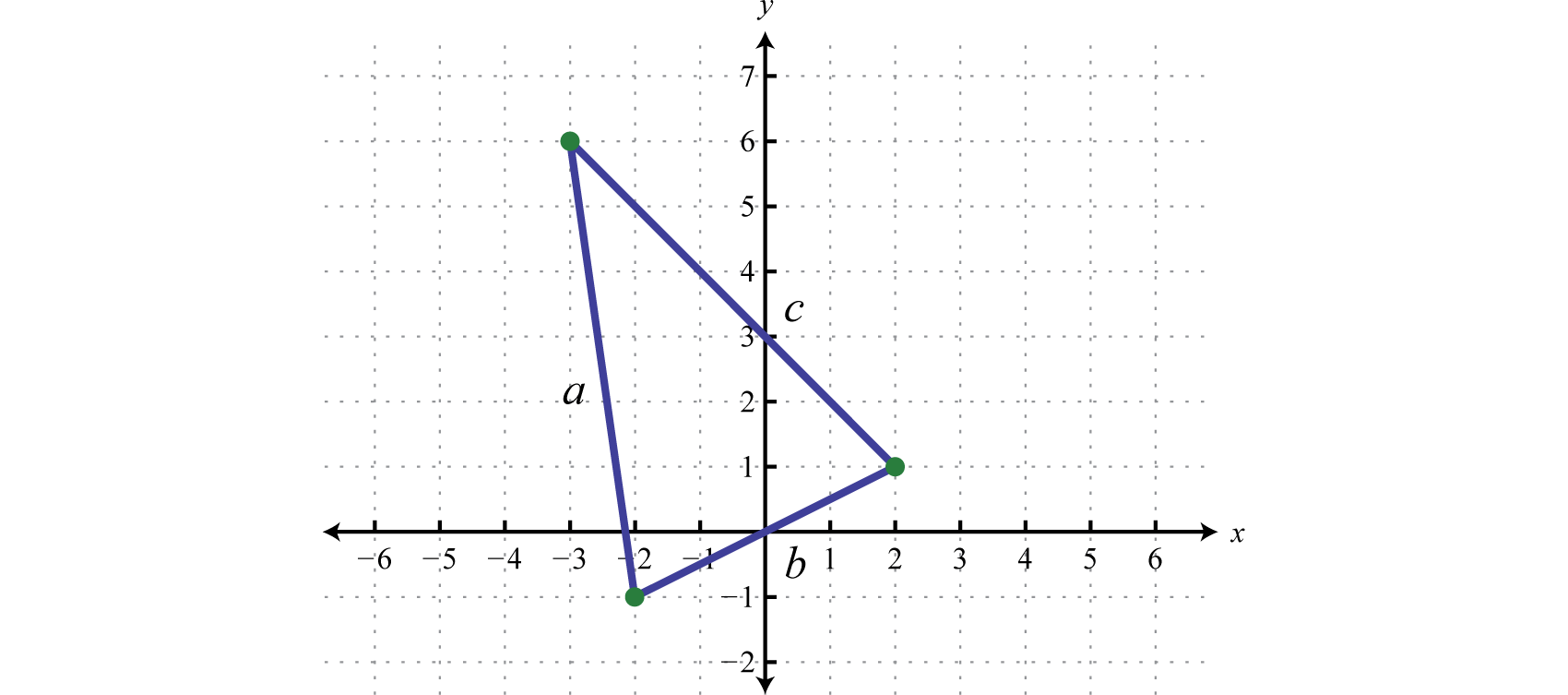Use the distance formula to calculate the length of each side.

$a=[−3−(−2)]2+[6−(−1)]2=(−3+2)2+(6+1)2=(−1)2+(7)2=1+49=50=52 b=[2−(−2)]2+[1−(−1)]2=(2+2)2+(1+1)2=(4)2+(2)2=16+4=20=25$

Similarly we can calculate the distance between (−3, 6) and (2,1) and find that $c=52$ units. Therefore, we can calculate the perimeter as follows:

$P=a+b+c=52+25+52=102+25$

Answer: $102+25$ units

### Key Takeaways

• Add and subtract terms that contain like radicals just as you do like terms. If the index and radicand are exactly the same, then the radicals are similar and can be combined. This involves adding or subtracting only the coefficients; the radical part remains the same.
• Simplify each radical completely before combining like terms.

### Topic Exercises

Simplify

1. $103−53$

2. $156−86$

3. $93+53$

4. $126+36$

5. $45−75−25$

6. $310−810−210$

7. $6−46+26$

8. $510−1510−210$

9. $137−62−57+52$

10. $1013−1215+513−1815$

11. $65−(43−35)$

12. $−122−(66+2)$

13. $(25−310)−(10+35)$

14. $(−83+615)−(3−15)$

15. $463−353+663$

16. $103+5103−4103$

17. $(793−433)−(93−333)$

18. $(−853+253)−(253+6253)$

Simplify. (Assume all radicands containing variable expressions are positive.)

1. $2x−42x$

2. $53y−63y$

3. $9x+7x$

4. $−8y+4y$

5. $7xy−3xy+xy$

6. $10y2x−12y2x−2y2x$

7. $2ab−5a+6ab−10a$

8. $−3xy+6y−4xy−7y$

9. $5xy−(3xy−7xy)$

10. $−8ab−(2ab−4ab)$

11. $(32x−3x)−(2x−73x)$

12. $(y−42y)−(y−52y)$

13. $5x3−12x3$

14. $−2y3−3y3$

15. $a3b5+4a3b5−a3b5$

16. $−8ab4+3ab4−2ab4$

17. $62a−42a3+72a−2a3$

18. $43a5+3a3−93a5+3a3$

19. $(4xy4−xy3)−(24xy4−xy3)$

20. $(56y6−5y)−(26y6+3y)$

21. $2x23x3−(x23x3−x3x3)$

22. $5y36y−(6y−4y36y)$

Simplify.

1. $75−12$

2. $24−54$

3. $32+27−8$

4. $20+48−45$

5. $28−27+63−12$

6. $90+24−40−54$

7. $45−80+245−5$

8. $108+48−75−3$

9. $42−(27−72)$

10. $−35−(20−50)$

11. $163−543$

12. $813−243$

13. $1353+403−53$

14. $1083−323−43$

15. $227−212$

16. $350−432$

17. $3243−218−48$

18. $6216−224−296$

19. $218−375−298+448$

20. $245−12+220−108$

21. $(2363−396)−(712−254)$

22. $(2288+3360)−(272−740)$

23. $3543+52503−4163$

24. $41623−23843−37503$

Simplify. (Assume all radicands containing variable expressions are positive.)

1. $81b+4b$

2. $100a+a$

3. $9a2b−36a2b$

4. $50a2−18a2$

5. $49x−9y+x−4y$

6. $9x+64y−25x−y$

7. $78x−(316y−218x)$

8. $264y−(332y−81y)$

9. $29m2n−5m9n+m2n$

10. $418n2m−2n8m+n2m$

11. $4x2y−9xy2−16x2y+y2x$

12. $32x2y2+12x2y−18x2y2−27x2y$

13. $(9x2y−16y)−(49x2y−4y)$

14. $(72x2y2−18x2y)−(50x2y2+x2y)$

15. $12m4n−m75m2n+227m4n$

16. $5n27mn2+212mn4−n3mn2$

17. $227a3b−a48ab−a144a3b$

18. $298a4b−2a162a2b+a200b$

19. $125a3−27a3$

20. $1000a23−64a23$

21. $2x54x3−216x43+52x43$

22. $x54x33−250x63+x223$

23. $16y24+81y24$

24. $32y45−y45$

25. $32a34−162a34+52a34$

26. $80a4b4+5a4b4−a5b4$

27. $27x33+8x3−125x33$

28. $24x3−128x3−81x3$

29. $27x4y3−8xy33+x64xy3−yx3$

30. $125xy33+8x3y3−216xy33+10xy3$

31. $(162x4y3−250x4y23)−(2x4y23−384x4y3)$
32. $(32x2y65−243x6y25)−(x2y65−xxy25)$

Calculate the perimeters of the triangles formed by the following sets of vertices.

1. {(−4, −5), (−4, 3), (2, 3)}

2. {(−1, 1), (3, 1), (3, −2)}

3. {(−3, 1), (−3, 5), (1, 5)}

4. {(−3, −1), (−3, 7), (1, −1)}

5. {(0,0), (2,4), (−2,6)}

6. {(−5,−2), (−3,0), (1,−6)}

7. A square garden that is 10 feet on each side is to be fenced in. In addition, the space is to be partitioned in half using a fence along its diagonal. How much fencing is needed to do this? (Round to the nearest tenth of a foot.)

8. A garden in the shape of a square has an area of 150 square feet. How much fencing is needed to fence it in? (Hint: The length of each side of a square is equal to the square root of the area. Round to the nearest tenth of a foot.)

### Part C: Discussion Board

1. Choose values for x and y and use a calculator to show that $x+y≠x+y.$

2. Choose values for x and y and use a calculator to show that $x2+y2≠x+y.$

1. $53$

2. $143$

3. $−55$

4. $−6$

5. $87−2$

6. $95−43$

7. $−5−410$

8. $1063−353$

9. $693−33$

10. $−32x$

11. $16x$

12. $5xy$

13. $8ab−15a$

14. $9xy$

15. $22x+63x$

16. $−7x3$

17. $4a3b5$

18. $132a−52a3$

19. $−4xy4$

20. $x23x3+x3x3$

1. $33$

2. $22+33$

3. $57−53$

4. $55$

5. $102−33$

6. $−23$

7. $453$

8. $23$

9. $233−62$

10. $−82+3$

11. $83−66$

12. $2623$

13. $11b$

14. $−3ab$

15. $8x−5y$

16. $202x−12y$

17. $−8mn$

18. $−2xy−2yx$

19. $−4xy$

20. $3m23n$

21. $2a3ab−12a2ab$

22. $2a3$

23. $7x2x3$

24. $5y24$

25. $42a34$

26. $−2x+2x3$

27. $7xxy3−3yx3$

28. $7x6xy3−6x2xy23$

29. 24 units

30. $8+42$ units

31. $45+210$ units

32. 54.1 feet

## 5.4 Multiplying and Dividing Radical Expressions

### Learning Objectives

3. Rationalize the denominator.

When multiplying radical expressions with the same index, we use the product rule for radicals. Given real numbers $An$ and $Bn$,

$An⋅Bn=A⋅Bn$

### Example 1

Multiply: $123⋅63.$

Solution:

Apply the product rule for radicals, and then simplify.

$123⋅63=12⋅63Multiply the radicands.=723Simplify.=23⋅323=2 323=2 93$

Answer: $293$

Often, there will be coefficients in front of the radicals.

### Example 2

Multiply: $36⋅52$

Solution:

Using the product rule for radicals and the fact that multiplication is commutative, we can multiply the coefficients and the radicands as follows.

$36⋅52=3⋅5 ⋅ 6⋅2Multiplication is commutative.=15 ⋅ 12Multiply the coefficients andthe radicands.=154⋅3 Simplify.=15⋅2⋅3=303$

Typically, the first step involving the application of the commutative property is not shown.

Answer: $303$

### Example 3

Multiply: $−34y23 ⋅ 516y3.$

Solution:

$−3 4y23⋅ 5 16y3=−15 64y33Multiply the coefficients and then multiply the radicands.=−15 43y33Simplify.=−15⋅4y=−60y$

Answer: $−60y$

Use the distributive property when multiplying rational expressions with more than one term.

### Example 4

Multiply: $52x(3x−2x)$.

Solution:

Apply the distributive property and multiply each term by $52x.$

$52x(3x−2x)=52x⋅3x−52x⋅2xDistribute.=152x2−54x2Simplify.=15x2−5⋅2x=15x2−10x$

Answer: $15x2−10x$

### Example 5

Multiply: $6x2y3( 9x2y23−5 ⋅4xy3).$

Solution:

Apply the distributive property, and then simplify the result.

$6x2y3( 9x2y23−5 ⋅4xy3)=6x2y3⋅ 9x2y23−6x2y3⋅ 5 4xy3 =54x4y33−5 24x3y23 =27⋅2⋅x⋅x3⋅y33−5 8⋅3⋅x3⋅y23 =3xy 2x3−5⋅2x 3y23=3xy 2x3−10x 3y23$

Answer: $3xy2x3−10x3y23$

The process for multiplying radical expressions with multiple terms is the same process used when multiplying polynomials. Apply the distributive property, simplify each radical, and then combine like terms.

### Example 6

Multiply: $(x−5y)2.$

Solution:

$(x−5y)2=(x−5y)(x−5y)$

Begin by applying the distributive property.$=x⋅x+x(−5y)+(−5y)x+(−5y)(−5y)=x2−5xy−5xy+25y2=x−10xy+25y$

Answer: $x−10xy+25y$

The binomials $(a+b)$ and $(a−b)$ are called conjugatesThe factors $(a+b)$ and $(a−b)$ are conjugates.. When multiplying conjugate binomials the middle terms are opposites and their sum is zero.

### Example 7

Multiply: $(10+3)(10−3).$

Solution:

Apply the distributive property, and then combine like terms.

$(10+3)(10−3)=10⋅10+10(−3)+3⋅10+3(−3)Distribute.=100−30+30−9Simplify.=10−30+30−3opposites add to 0=10 − 3=7$

It is important to note that when multiplying conjugate radical expressions, we obtain a rational expression. This is true in general

$(x+y)(x−y)=x2−xy+xy−y2=x−y$

Alternatively, using the formula for the difference of squares we have,

$(a+b)(a−b)=a2−b2Difference of squares.(x+y)(x−y)=(x)2−(y)2=x−y$

Try this! Multiply: $(3−2y)(3+2y).$ (Assume y is positive.)

Answer: $9−4y$

To divide radical expressions with the same index, we use the quotient rule for radicals. Given real numbers $An$ and $Bn$,

$AnBn=ABn$

### Example 8

Divide: $96363$.

Solution:

In this case, we can see that 6 and 96 have common factors. If we apply the quotient rule for radicals and write it as a single cube root, we will be able to reduce the fractional radicand.

$96363=9663Apply the quotient rule for radicals and reduce the radicand.=163Simplify.=8⋅23=2 23$

Answer: $223$

### Example 9

Divide: $50x6y48x3y$.

Solution:

Write as a single square root and cancel common factors before simplifying.

$50x6y48x3y=50x6y48x3yApply the quotient rule for radicals and cancel.=25x3y34Simplify.=25x3y34=5xyxy2$

Answer: $5xyxy2$

## Rationalizing the Denominator

When the denominator (divisor) of a radical expression contains a radical, it is a common practice to find an equivalent expression where the denominator is a rational number. Finding such an equivalent expression is called rationalizing the denominatorThe process of determining an equivalent radical expression with a rational denominator..

$Radical expressionRational denominator12=22$

To do this, multiply the fraction by a special form of 1 so that the radicand in the denominator can be written with a power that matches the index. After doing this, simplify and eliminate the radical in the denominator. For example:

$12=12⋅22=24=22$

Remember, to obtain an equivalent expression, you must multiply the numerator and denominator by the exact same nonzero factor.

### Example 10

Rationalize the denominator: $25x.$

Solution:

The goal is to find an equivalent expression without a radical in the denominator. The radicand in the denominator determines the factors that you need to use to rationalize it. In this example, multiply by 1 in the form $5x5x.$

$25x=25x⋅5x5x Multiply by 5x5x.=10x25x2Simplify.=10x5x$

Answer: $10x5x$

Sometimes, we will find the need to reduce, or cancel, after rationalizing the denominator.

### Example 11

Rationalize the denominator: $3a26ab.$

Solution:

In this example, we will multiply by 1 in the form $6ab6ab.$

$3a26ab=3a26ab⋅6ab6ab =3a12ab36a2b2Simplify.=3a4⋅3ab6ab =6a3ab6abCancel.=3abb$

Notice that b does not cancel in this example. Do not cancel factors inside a radical with those that are outside.

Answer: $3abb$

Try this! Rationalize the denominator: $9x2y.$

Answer: $32xy2y$

Up to this point, we have seen that multiplying a numerator and a denominator by a square root with the exact same radicand results in a rational denominator. In general, this is true only when the denominator contains a square root. However, this is not the case for a cube root. For example, $1x3⋅x3x3=x3x23$ Note that multiplying by the same factor in the denominator does not rationalize it. In this case, if we multiply by 1 in the form of $x23x23$, then we can write the radicand in the denominator as a power of 3. Simplifying the result then yields a rationalized denominator.

$1x3=1x3⋅x23x23=x23x33=x23x$

Therefore, to rationalize the denominator of a radical expression with one radical term in the denominator, begin by factoring the radicand of the denominator. The factors of this radicand and the index determine what we should multiply by. Multiply the numerator and denominator by the nth root of factors that produce nth powers of all the factors in the radicand of the denominator.

### Example 12

Rationalize the denominator: $23253.$

Solution:

The radical in the denominator is equivalent to $523.$ To rationalize the denominator, we need: $533.$ To obtain this, we need one more factor of 5. Therefore, multiply by 1 in the form of $5353.$

$23253=23523⋅5353Multiply by the cube root of factors that result in powers of 3.=103533Simplify.=1035$

Answer: $1035$

### Example 13

Rationalize the denominator: $27a2b23$.

Solution:

In this example, we will multiply by 1 in the form $22b322b3$.

$27a2b23=33a32b23Apply the quotient rule for radicals.=3a32b23⋅22b322b3Multiply by the cube root of factors that result in powers of 3. =322ab323b33Simplify.=34ab32b$

Answer: $34ab32b$

### Example 14

Rationalize the denominator: $2x55 4x3y5$.

Solution:

In this example, we will multiply by 1 in the form $23x2y4523x2y45$.

$2x554x3y5=2x5522x3y5⋅23x2y4523x2y45Multiply by the fifth root of factors that result in powers of 5. =2x5⋅23x2y4525x5y55Simplify.=2x40x2y452xy=40x2y45y$

Answer: $40x2y45y$

When two terms involving square roots appear in the denominator, we can rationalize it using a very special technique. This technique involves multiplying the numerator and the denominator of the fraction by the conjugate of the denominator. Recall that multiplying a radical expression by its conjugate produces a rational number.

### Example 15

Rationalize the denominator: $15−3.$

Solution:

In this example, the conjugate of the denominator is $5+3.$ Therefore, multiply by 1 in the form $(5+3)(5+3).$

$15−3=1(5−3) (5+3)(5+3)Multiply numerator anddenominator by the conjugate of the denominator.=5+325+15−15−9Simplify.=5+35−3=5+32$

Answer: $5+32$

Notice that the terms involving the square root in the denominator are eliminated by multiplying by the conjugate. We can use the property $(a+b)(a−b)=a−b$ to expedite the process of multiplying the expressions in the denominator.

### Example 16

Rationalize the denominator: $102+6$.

Solution:

Multiply by 1 in the form $2−62−6$.

$102+6=(10)(2+6)(2−6)(2−6)Multiply by the conjugate of the denominator.=20−602−6Simplify.=4⋅5−4⋅15−4=25−215−4=2(5−15)−4=5−15−2=−5−152=−5+152$

Answer: $15−52$

### Example 17

Rationalize the denominator: $x−yx+y$.

Solution:

In this example, we will multiply by 1 in the form $x−yx−y.$

$x−yx+y=(x−y)(x+y)(x−y)(x−y)Multiply by the conjugate of the denominator.=x2−xy−xy+y2x−ySimplify.=x−2xy+yx−y$

Answer: $x−2xy+yx−y$

Try this! Rationalize the denominator: $235−3$

Answer: $53+311$

### Key Takeaways

• To multiply two single-term radical expressions, multiply the coefficients and multiply the radicands. If possible, simplify the result.
• Apply the distributive property when multiplying a radical expression with multiple terms. Then simplify and combine all like radicals.
• Multiplying a two-term radical expression involving square roots by its conjugate results in a rational expression.
• It is common practice to write radical expressions without radicals in the denominator. The process of finding such an equivalent expression is called rationalizing the denominator.
• If an expression has one term in the denominator involving a radical, then rationalize it by multiplying the numerator and denominator by the nth root of factors of the radicand so that their powers equal the index.
• If a radical expression has two terms in the denominator involving square roots, then rationalize it by multiplying the numerator and denominator by the conjugate of the denominator.

### Part A: Multiplying Radical Expressions

Multiply. (Assume all variables represent non-negative real numbers.)

1. $3⋅7$

2. $2⋅5$

3. $6⋅12$

4. $10⋅15$

5. $2⋅6$

6. $5⋅15$

7. $7⋅7$

8. $12⋅12$

9. $25⋅710$

10. $315⋅26$

11. $(25)2$

12. $(62)2$

13. $2x⋅2x$

14. $5y⋅5y$

15. $3a⋅12$

16. $3a⋅2a$

17. $42x⋅36x$

18. $510y⋅22y$

19. $33⋅93$

20. $43⋅163$

21. $153⋅253$

22. $1003⋅503$

23. $43⋅103$

24. $183⋅63$

25. $(593)(263)$

26. $(243)(343)$

27. $(223)3$

28. $(343)3$

29. $3a23⋅9a3$

30. $7b3⋅49b23$

31. $6x23⋅4x23$

32. $12y3⋅9y23$

33. $20x2y3⋅10x2y23$

34. $63xy3⋅12x4y23$

35. $5(3−5)$

36. $2(3−2)$

37. $37(27−3)$

38. $25(6−310)$

39. $6(3−2)$

40. $15(5+3)$

41. $x(x+xy)$

42. $y(xy+y)$

43. $2ab(14a−210b)$

44. $6ab(52a−3b)$

45. $63(93−203)$

46. $123(363+143)$

47. $(2−5)(3+7)$

48. $(3+2)(5−7)$

49. $(23−4)(36+1)$

50. $(5−26)(7−23)$

51. $(5−3)2$

52. $(7−2)2$

53. $(23+2)(23−2)$

54. $(2+37)(2−37)$

55. $(a−2b)2$

56. $(ab+1)2$

57. What is the perimeter and area of a rectangle with length measuring $53$ centimeters and width measuring $32$ centimeters?

58. What is the perimeter and area of a rectangle with length measuring $26$ centimeters and width measuring $3$ centimeters?

59. If the base of a triangle measures $62$ meters and the height measures $32$ meters, then calculate the area.

60. If the base of a triangle measures $63$ meters and the height measures $36$ meters, then calculate the area.

### Part B: Dividing Radical Expressions

Divide. (Assume all variables represent positive real numbers.)

1. $753$
2. $36010$
3. $7275$
4. $9098$
5. $90x52x$
6. $96y33y$
7. $162x7y52xy$
8. $363x4y93xy$
9. $16a5b232a2b23$
10. $192a2b732a2b23$

### Part C: Rationalizing the Denominator

Rationalize the denominator. (Assume all variables represent positive real numbers.)

1. $15$
2. $16$
3. $23$
4. $37$
5. $5210$
6. $356$
7. $3−53$
8. $6−22$
9. $17x$
10. $13y$
11. $a5ab$
12. $3b223ab$
13. $2363$
14. $1473$
15. $14x3$
16. $13y23$
17. $9x239xy23$
18. $5y2x35x2y3$
19. $3a23a2b23$
20. $25n325m2n3$
21. $327x2y5$
22. $216xy25$
23. $ab9a3b5$
24. $abcab2c35$
25. $3x8y2z5$
26. $4xy29x3yz45$
27. $310−3$
28. $26−2$
29. $15+3$
30. $17−2$
31. $33+6$
32. $55+15$
33. $105−35$
34. $−224−32$
35. $3+53−5$
36. $10−210+2$
37. $23−3243+2$
38. $65+225−2$
39. $x−yx+y$
40. $x−yx−y$
41. $x+yx−y$
42. $x−yx+y$
43. $a−ba+b$
44. $ab+2ab−2$
45. $x5−2x$
46. $1x−y$
47. $x+2y2x−y$
48. $3x−yx+3y$
49. $2x+12x+1−1$
50. $x+11−x+1$
51. $x+1+x−1x+1−x−1$
52. $2x+3−2x−32x+3+2x−3$
53. The radius of the base of a right circular cone is given by $r=3Vπh$ where V represents the volume of the cone and h represents its height. Find the radius of a right circular cone with volume 50 cubic centimeters and height 4 centimeters. Give the exact answer and the approximate answer rounded to the nearest hundredth.

54. The radius of a sphere is given by $r=3V4π3$ where V represents the volume of the sphere. Find the radius of a sphere with volume 135 square centimeters. Give the exact answer and the approximate answer rounded to the nearest hundredth.

### Part D: Discussion

1. Research and discuss some of the reasons why it is a common practice to rationalize the denominator.

2. Explain in your own words how to rationalize the denominator.

1. $21$

2. $62$

3. $23$

4. 7

5. $702$

6. 20

7. $2x$

8. $6a$

9. $24x3$

10. 3

11. $533$

12. $253$

13. $3023$

14. 16

15. $3a$

16. $2x3x3$

17. $2xy25x3$

18. $35−5$

19. $42−321$

20. $32−23$

21. $x+xy$

22. $2a7b−4b5a$

23. $323−2153$

24. $6+14−15−35$

25. $182+23−126−4$

26. $8−215$

27. 10

28. $a−22ab+2b$

29. Perimeter: $(103+62)$ centimeters; area: $156$ square centimeters

30. $18$ square meters

1. 5

2. $265$
3. $3x25$

4. $9x3y2$

5. $2a$

1. $55$
2. $63$
3. $104$
4. $3−153$
5. $7x7x$
6. $ab5b$
7. $633$
8. $2x232x$
9. $36x2y3y$
10. $9ab32b$
11. $9x3y45xy$
12. $27a2b453$
13. $12xy3z452yz$
14. $310+9$

15. $5−32$
16. $−1+2$

17. $−5−352$
18. $−4−15$

19. $15−7623$
20. $x−y$

21. $x2+2xy+yx2−y$
22. $a−2ab+ba−b$
23. $5x+2x25−4x$
24. $x2+3xy+y22x−y$
25. $2x+1+2x+12x$
26. $x+x2−1$

27. $56π2π$ centimeters; 3.45 centimeters

## 5.5 Rational Exponents

### Learning Objectives

1. Write expressions with rational exponents in radical form.
2. Write radical expressions with rational exponents.
3. Perform operations and simplify expressions with rational exponents.
4. Perform operations on radicals with different indices.

## Rational Exponents

So far, exponents have been limited to integers. In this section, we will define what rational (or fractional) exponents mean and how to work with them. All of the rules for exponents developed up to this point apply. In particular, recall the product rule for exponents. Given any rational numbers m and n, we have $xm⋅xn=xm+n$ For example, if we have an exponent of 1/2, then the product rule for exponents implies the following: $51/2⋅51/2=51/2+1/2=51=5$ Here $51/2$ is one of two equal factors of 5; hence it is a square root of 5, and we can write $51/2=5$ Furthermore, we can see that $21/3$ is one of three equal factors of 2. $21/3⋅21/3⋅21/3=21/3+1/3+1/3=23/3=21=2$ Therefore, $21/3$ is a cube root of 2, and we can write $21/3=23$ This is true in general, given any nonzero real number a and integer $n≥2$, $a1/n=an$ In other words, the denominator of a fractional exponent determines the index of an nth root.

### Example 1

1. $61/2$
2. $61/3$

Solution:

1. $61/2=62=6$
2. $61/3=63$

### Example 2

Rewrite as a radical and then simplify.

1. $161/2$
2. $161/4$

Solution:

1. $161/2=16=42=4$
2. $161/4=164=244=2$

### Example 3

Rewrite as a radical and then simplify.

1. $(64x3)1/3$
2. $(−32x5y10)1/5$

Solution:

a.

$(64x3)1/3=64x33=43x33=4x$

b.

$(−32x5y10)1/5=−32x5y105=(−2)5x5(y2)55=−2xy2$

Next, consider fractional exponents where the numerator is an integer other than 1. For example, consider the following:

$52/3⋅52/3⋅52/3=52/3+2/3+2/3=56/3=52$

This shows that $52/3$ is one of three equal factors of $52.$ In other words, $52/3$ is a cube root of $52$ and we can write:

$52/3=523$

In general, given any nonzero real number a where m and n are positive integers ($n≥2$),

$am/n=amn$

An expression with a rational exponentThe fractional exponent m/n that indicates a radical with index n and exponent m: $am/n=amn.$ is equivalent to a radical where the denominator is the index and the numerator is the exponent. Any radical expression can be written with a rational exponent, which we call exponential formAn equivalent expression written using a rational exponent..

$Radical formExponential formx25=x2/5$

### Example 4

1. $62/5$
2. $33/4$

Solution:

1. $62/5=625=365$
2. $33/4=334=274$

### Example 5

Rewrite as a radical and then simplify.

1. $272/3$
2. $(12)5/3$

Solution:

We can often avoid very large integers by working with their prime factorization.

a.

$272/3=2723=(33)23Replace 27 with 33.=363Simplify.=32=9$

b.

$(12)5/3=(12)53Replace 12 with 22⋅3.=(22⋅3)53Apply the rules for exponents.=210⋅353Simplify.=29⋅2⋅33⋅323=23⋅3⋅2⋅323=24 183$

Given a radical expression, we might want to find the equivalent in exponential form. Assume all variables are positive.

### Example 6

Rewrite using rational exponents: $x35.$

Solution:

Here the index is 5 and the power is 3. We can write

$x35=x3/5$

Answer: $x3/5$

### Example 7

Rewrite using rational exponents: $y36.$

Solution:

Here the index is 6 and the power is 3. We can write

$y36=y3/6=y1/2$

Answer: $y1/2$

It is important to note that the following are equivalent.

$am/n=amn=(an)m$

In other words, it does not matter if we apply the power first or the root first. For example, we can apply the power before the nth root:

$272/3=2723=(33)23=363=32=9$

Or we can apply the nth root before the power:

$272/3=(273)2=(333)2=(3)2=9$

The results are the same.

### Example 8

Rewrite as a radical and then simplify: $(−8)2/3.$

Solution:

Here the index is 3 and the power is 2. We can write

$(−8)2/3=(−83)2=(−2)2=4$

Try this! Rewrite as a radical and then simplify: $1003/2.$

Some calculators have a caret button $^$ which is used for entering exponents. If so, we can calculate approximations for radicals using it and rational exponents. For example, to calculate $2=21/2=2^(1/2)≈1.414$, we make use of the parenthesis buttons and type

$2 ^ ( 1 ÷ 2 ) =$

To calculate $223=22/3=2^(2/3)≈1.587$, we would type

$2 ^ ( 2 ÷ 3 ) =$

## Operations Using the Rules of Exponents

In this section, we review all of the rules of exponents, which extend to include rational exponents. If given any rational numbers m and n, then we have

 Product rule for exponents: $xm⋅xn=xm+n$ Quotient rule for exponents: $xmxn=xm−n, x≠0$ Power rule for exponents: $(xm)n=xm⋅n$ Power rule for a product: $(xy)n=xnyn$ Power rule for a quotient: $(xy)n=xnyn , y≠0$ Negative exponents: $x−n=1xn$ Zero exponent: $x0=1, x≠0$

These rules allow us to perform operations with rational exponents.

### Example 9

Simplify: $71/3⋅74/9.$

Solution:

$71/3⋅74/9=71/3+4/9Apply the product rule xm⋅xn=xm+n.=73/9+4/9=77/9$

Answer: $77/9$

### Example 10

Simplify: $x3/2x2/3$.

Solution:

$x3/2x2/3=x3/2−2/3Apply the quotient rule xmxn=xm−n .=x9/6−4/6=x5/6$

Answer: $x5/6$

### Example 11

Simplify: $(y3/4)2/3.$

Solution:

$(y3/4)2/3=y(3/4)(2/3)Apply the power rule (xm)n=xm⋅n .=y6/12 Multiply the exponents and reduce.=y1/2$

Answer: $y1/2$

### Example 12

Simplify: $(81a8b12)3/4$.

Solution:

$(81a8b12)3/4=(34a8b12)3/4Rewrite 81 as 34.=(34)3/4(a8)3/4(b12)3/4Apply the power rule for a product (xy)n=xnyn .=34(3/4)a8(3/4)b12(3/4)Apply the power rule to each factor.=33a6b9Simplify.=27a6b9$

Answer: $27a6b9$

### Example 13

Simplify: $(9x4)−3/2.$

Solution:

$(9x4)−3/2=1(9x4)3/2Apply the definition of negative exponents x−n=1xn.=1(32x4)3/2Write 9 as 32 and apply the rules of exponents.=132(3/2)x4(3/2)=133⋅x6=127x6$

Answer: $127x6$

Try this! Simplify: $(125a1/4b6)2/3a1/6$.

Answer: $25b4$

## Radical Expressions with Different Indices

To apply the product or quotient rule for radicals, the indices of the radicals involved must be the same. If the indices are different, then first rewrite the radicals in exponential form and then apply the rules for exponents.

### Example 14

Multiply: $2⋅23.$

Solution:

In this example, the index of each radical factor is different. Hence the product rule for radicals does not apply. Begin by converting the radicals into an equivalent form using rational exponents. Then apply the product rule for exponents.

$2⋅23=21/2⋅21/3Equivalents using rational exponents=21/2+1/3Apply the product rule for exponents.=25/6=256$

Answer: $256$

### Example 15

Divide: $4325$.

Solution:

In this example, the index of the radical in the numerator is different from the index of the radical in the denominator. Hence the quotient rule for radicals does not apply. Begin by converting the radicals into an equivalent form using rational exponents and then apply the quotient rule for exponents.

$4325=22325=22/321/5Equivalents using rational exponents=22/3−1/5Apply the quotient rule for exponents.=27/15=2715$

Answer: $2715$

### Example 16

Simplify: $43 .$

Solution:

Here the radicand of the square root is a cube root. After rewriting this expression using rational exponents, we will see that the power rule for exponents applies.

$43 = 223 =(22/3)1/2Equivalents using rational exponents=2(2/3)(1/2)Apply the power rule for exponents.=21/3=23$

Answer: $23$

### Key Takeaways

• Any radical expression can be written in exponential form: $amn=am/n.$
• Fractional exponents indicate radicals. Use the numerator as the power and the denominator as the index of the radical.
• All the rules of exponents apply to expressions with rational exponents.
• If operations are to be applied to radicals with different indices, first rewrite the radicals in exponential form and then apply the rules for exponents.

### Part A: Rational Exponents

Express using rational exponents.

1. $10$

2. $6$

3. $33$

4. $54$

5. $523$

6. $234$

7. $493$

8. $93$

9. $x5$

10. $x6$

11. $x76$

12. $x45$

13. $1x$
14. $1x23$

1. $101/2$

2. $111/3$

3. $72/3$

4. $23/5$

5. $x3/4$

6. $x5/6$

7. $x−1/2$

8. $x−3/4$

9. $(1x)−1/3$
10. $(1x)−3/5$
11. $(2x+1)2/3$

12. $(5x−1)1/2$

Write as a radical and then simplify.

1. $641/2$

2. $491/2$

3. $(14)1/2$
4. $(49)1/2$
5. $4−1/2$

6. $9−1/2$

7. $(14)−1/2$
8. $(116)−1/2$
9. $81/3$

10. $1251/3$

11. $(127)1/3$
12. $(8125)1/3$
13. $(−27)1/3$

14. $(−64)1/3$

15. $161/4$

16. $6251/4$

17. $81−1/4$

18. $16−1/4$

19. $100,0001/5$

20. $(−32)1/5$

21. $(132)1/5$
22. $(1243)1/5$
23. $93/2$

24. $43/2$

25. $85/3$

26. $272/3$

27. $163/2$

28. $322/5$

29. $(116)3/4$
30. $(181)3/4$
31. $(−27)2/3$

32. $(−27)4/3$

33. $(−32)3/5$

34. $(−32)4/5$

Use a calculator to approximate an answer rounded to the nearest hundredth.

1. $21/2$

2. $21/3$

3. $23/4$

4. $32/3$

5. $51/5$

6. $71/7$

7. $(−9)3/2$

8. $−93/2$

9. Explain why (−4)^(3/2) gives an error on a calculator and −4^(3/2) gives an answer of −8.

10. Marcy received a text message from Mark asking her age. In response, Marcy texted back “125^(2/3) years old.” Help Mark determine Marcy’s age.

### Part B: Operations Using the Rules of Exponents

Perform the operations and simplify. Leave answers in exponential form.

1. $53/2⋅51/2$

2. $32/3⋅37/3$

3. $51/2⋅51/3$

4. $21/6⋅23/4$

5. $y1/4⋅y2/5$

6. $x1/2⋅x1/4$

7. $511/352/3$
8. $29/221/2$
9. $2a2/3a1/6$
10. $3b1/2b1/3$
11. $(81/2)2/3$
12. $(36)2/3$
13. $(x2/3)1/2$
14. $(y3/4)4/5$
15. $(y8)−1/2$
16. $(y6)−2/3$
17. $(4x2y4)1/2$
18. $(9x6y2)1/2$
19. $(2x1/3y2/3)3$
20. $(8x3/2y1/2)2$
21. $(36x4y2)−1/2$
22. $(8x3y6z−3)−1/3$
23. $(a3/4a1/2)4/3$
24. $(b4/5b1/10)10/3$
25. $(4x2/3y4)1/2$
26. $(27x3/4y9)1/3$
27. $y1/2y2/3y1/6$
28. $x2/5x1/2x1/10$
29. $xyx1/2y1/3$
30. $x5/4yxy2/5$
31. $49a5/7b3/27a3/7b1/4$
32. $16a5/6b5/48a1/2b2/3$
33. $(9x2/3y6)3/2x1/2y$
34. $(125x3y3/5)2/3xy1/3$
35. $(27a1/4b3/2)2/3a1/6b1/2$
36. $(25a2/3b4/3)3/2a1/6b1/3$
37. $(16x2y−1/3z2/3)−3/2$

38. $(81x8y−4/3z−4)−3/4$

39. $(100a−2/3b4c−3/2)−1/2$

40. $(125a9b−3/4c−1)−1/3$

### Part C: Radical Expressions with Different Indices

Perform the operations.

1. $93⋅35$

2. $5⋅255$

3. $x⋅x3$

4. $y⋅y4$

5. $x23⋅x4$

6. $x35⋅x3$

7. $100310$
8. $16543$
9. $a23a$
10. $b45b3$
11. $x23x35$
12. $x34x23$
13. $165$

14. $93$

15. $253$

16. $553$

17. $73$

18. $33$

### Part D: Discussion Board

1. Who is credited for devising the notation that allows for rational exponents? What are some of his other accomplishments?

2. When using text, it is best to communicate nth roots using rational exponents. Give an example.

1. $101/2$

2. $31/3$

3. $52/3$

4. $72/3$

5. $x1/5$

6. $x7/6$

7. $x−1/2$

8. $10$

9. $493$

10. $x34$

11. $1x$
12. $x3$

13. $(2x+1)23$

14. 8

15. $12$

16. $12$

17. 2

18. 2

19. $13$

20. −3

21. 2

22. $13$

23. 10

24. $12$

25. 27

26. 32

27. 64

28. $18$

29. 9

30. −8

31. 1.41

32. 1.68

33. 1.38

34. Not a real number

1. 25

2. $55/6$

3. $y13/20$

4. 125

5. $2a1/2$

6. 2

7. $x1/3$

8. $1y4$
9. $2xy2$

10. $8xy2$

11. $16x2y$
12. $a1/3$

13. $2x1/3y2$
14. $y$

15. $x1/2y2/3$

16. $7a2/7b5/4$

17. $27x1/2y8$

18. $9b1/2$

19. $y1/264x3z$
20. $a1/3b3/410b2$
1. $31315$

2. $x56$

3. $x1112$

4. $106$

5. $a6$

6. $x15$

7. $45$

8. $215$

9. $76$

### Learning Objectives

1. Solve equations involving square roots.
2. Solve equations involving cube roots.

A radical equationAny equation that contains one or more radicals with a variable in the radicand. is any equation that contains one or more radicals with a variable in the radicand. Following are some examples of radical equations, all of which will be solved in this section:

 $2x−1=3$ $4x2+73−2=0$ $x+2−x=1$

We begin with the squaring property of equalityGiven real numbers a and b, where $a=b$, then $a2=b2.$; given real numbers a and b, we have the following:

$If a=b, then a2=b2.$

In other words, equality is retained if we square both sides of an equation.

$−3=−3⇒(−3)2=(−3)29=9 ✓$

The converse, on the other hand, is not necessarily true,

$9=9(−3)2=(3)2⇒−3≠3 ✗$

This is important because we will use this property to solve radical equations. Consider a very simple radical equation that can be solved by inspection,

$x=5$

Here we can see that $x=25$ is a solution. To solve this equation algebraically, make use of the squaring property of equality and the fact that $(a)2=a2=a$ when a is nonnegative. Eliminate the square root by squaring both sides of the equation as follows:

$(x)2=(5)2x=25$

As a check, we can see that $25=5$ as expected. Because the converse of the squaring property of equality is not necessarily true, solutions to the squared equation may not be solutions to the original. Hence squaring both sides of an equation introduces the possibility of extraneous solutionsA properly found solution that does not solve the original equation., which are solutions that do not solve the original equation. For example,

$x=−5$

This equation clearly does not have a real number solution. However, squaring both sides gives us a solution:

$(x)2=(−5)2x=25$

As a check, we can see that $25≠−5.$ For this reason, we must check the answers that result from squaring both sides of an equation.

### Example 1

Solve: $3x+1=4.$

Solution:

We can eliminate the square root by applying the squaring property of equality.

$3x+1=4(3x+1)2=(4)2Square both sides.3x+1=16Solve.3x=15x=5$

Next, we must check.

$3(5)+1=415+1=416=44=4 ✓$

There is a geometric interpretation to the previous example. Graph the function defined by $f(x)=3x+1$ and determine where it intersects the graph defined by $g(x)=4.$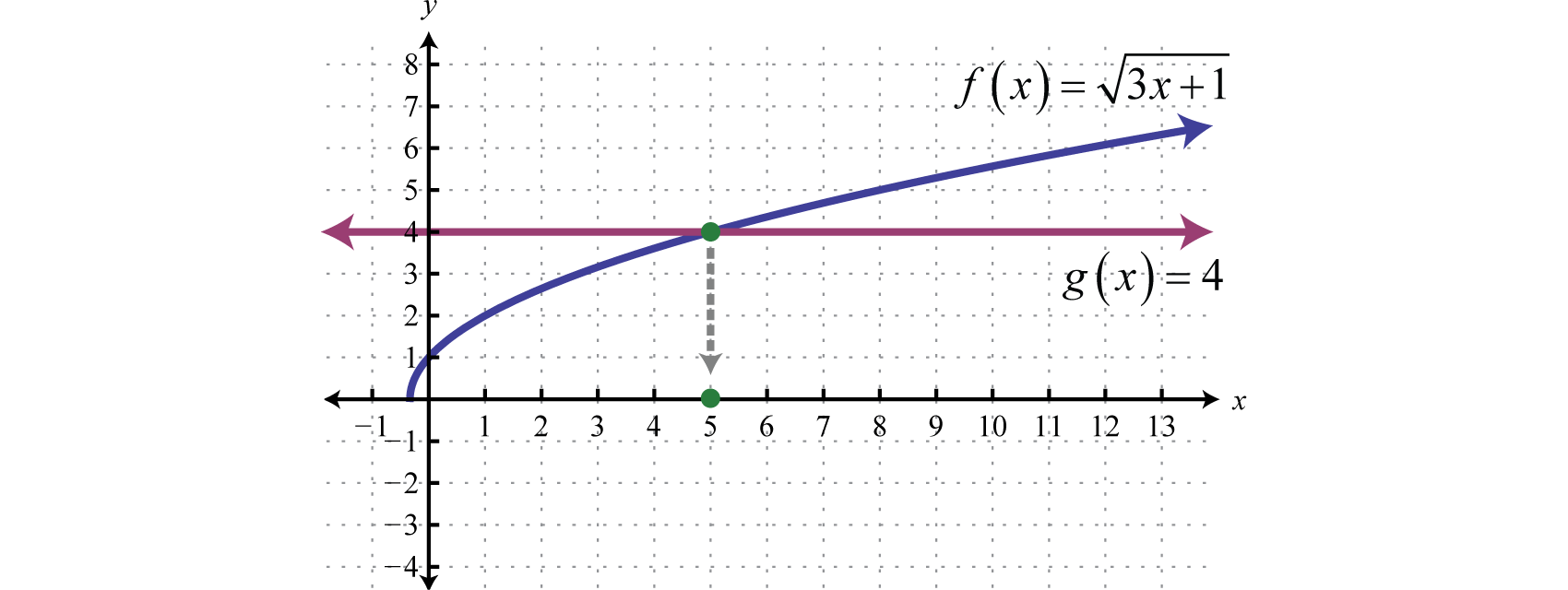As illustrated, $f(x)=g(x)$ where $x=5.$

### Example 2

Solve: $x−3=x−5.$

Solution:

Begin by squaring both sides of the equation.

$x−3=x−5(x−3)2=(x−5)2Square both sides.x−3=x2−10x+25$

The resulting quadratic equation can be solved by factoring.

$x−3=x2−10x+250=x2−11x+280=(x−4)(x−7)x−4=0or x−7=0x=4x=7$

Checking the solutions after squaring both sides of an equation is not optional. Use the original equation when performing the check.

$Check x=4$

$Check x=7$

$x−3=x−54−3=4−51=−11=−1 ✗$

$x−3=x−57−3=7−54=22=2 ✓$

After checking, you can see that $x=4$ is an extraneous solution; it does not solve the original radical equation. Disregard that answer. This leaves $x=7$ as the only solution.

Geometrically we can see that $f(x)=x+3$ is equal to $g(x)=x−5$ where $x=7.$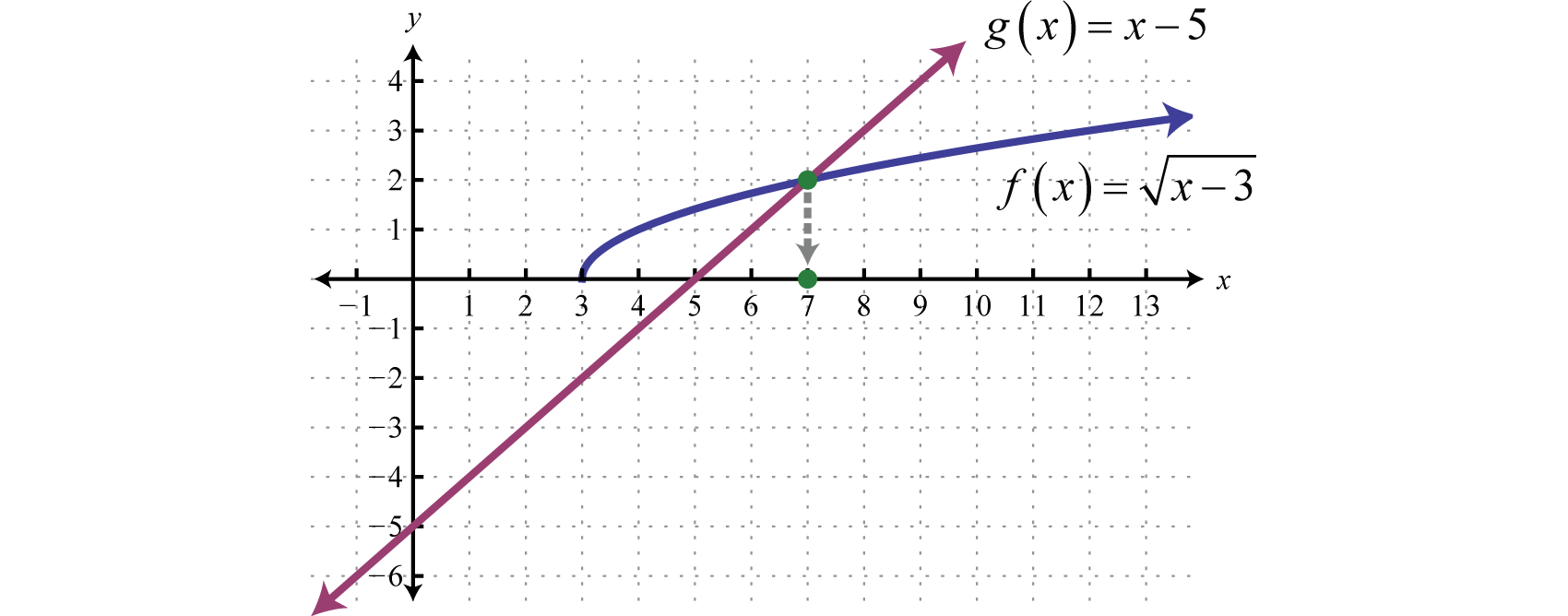In the previous two examples, notice that the radical is isolated on one side of the equation. Typically, this is not the case. The steps for solving radical equations involving square roots are outlined in the following example.

### Example 3

Solve: $2x−1+2=x.$

Solution:

Step 1: Isolate the square root. Begin by subtracting 2 from both sides of the equation.

$2x−1+2=x2x−1=x−2$

Step 2: Square both sides. Squaring both sides eliminates the square root.

$(2x−1)2=(x−2)22x−1=x2−4x+4$

Step 3: Solve the resulting equation. Here we are left with a quadratic equation that can be solved by factoring.

$2x−1=x2−4x+40=x2−6x+50=(x−1)(x−5)x−1=0or x−5=0x=1x=5$

Step 4: Check the solutions in the original equation. Squaring both sides introduces the possibility of extraneous solutions; hence the check is required.

$Check x=1$

$Check x=5$

$2x−1+2=x2(1)−1+2=11+2=11+2=13=1 ✗$

$2x−1+2=x2(5)−1+2=59+2=53+2=55=5 ✓$

After checking, we can see that $x=1$ is an extraneous solution; it does not solve the original radical equation. This leaves $x=5$ as the only solution.

Sometimes there is more than one solution to a radical equation.

### Example 4

Solve: $22x+5−x=4.$

Solution:

Begin by isolating the term with the radical.

$22x+5−x=4Add x to both sides.22x+5=x+4$

Despite the fact that the term on the left side has a coefficient, we still consider it to be isolated. Recall that terms are separated by addition or subtraction operators.

$22x+5=x+4(22x+5)2=(x+4)2Square both sides.4(2x+5)=x2+8x+16$

$4(2x+5)=x2+8x+168x+20=x2+8x+160=x2−40=(x+2)(x−2)x+2=0or x−2=0x=−2x=2$

Since we squared both sides, we must check our solutions.

$Check x=−2$

$Check x=2$

$22x+5−x=422(−2)+5−(−2)=42−4+5+2=421+2=42+2=44=4 ✓$

$22x+5−x=422(2)+5−(2)=424+5−2=429−2=46−2=44=4 ✓$

After checking, we can see that both are solutions to the original equation.

Solve: $4−11x−x+2=0.$
$4−11x−x+2=0Isolate the radical.4−11x=x−2(4−11x)2=(x−2)2$